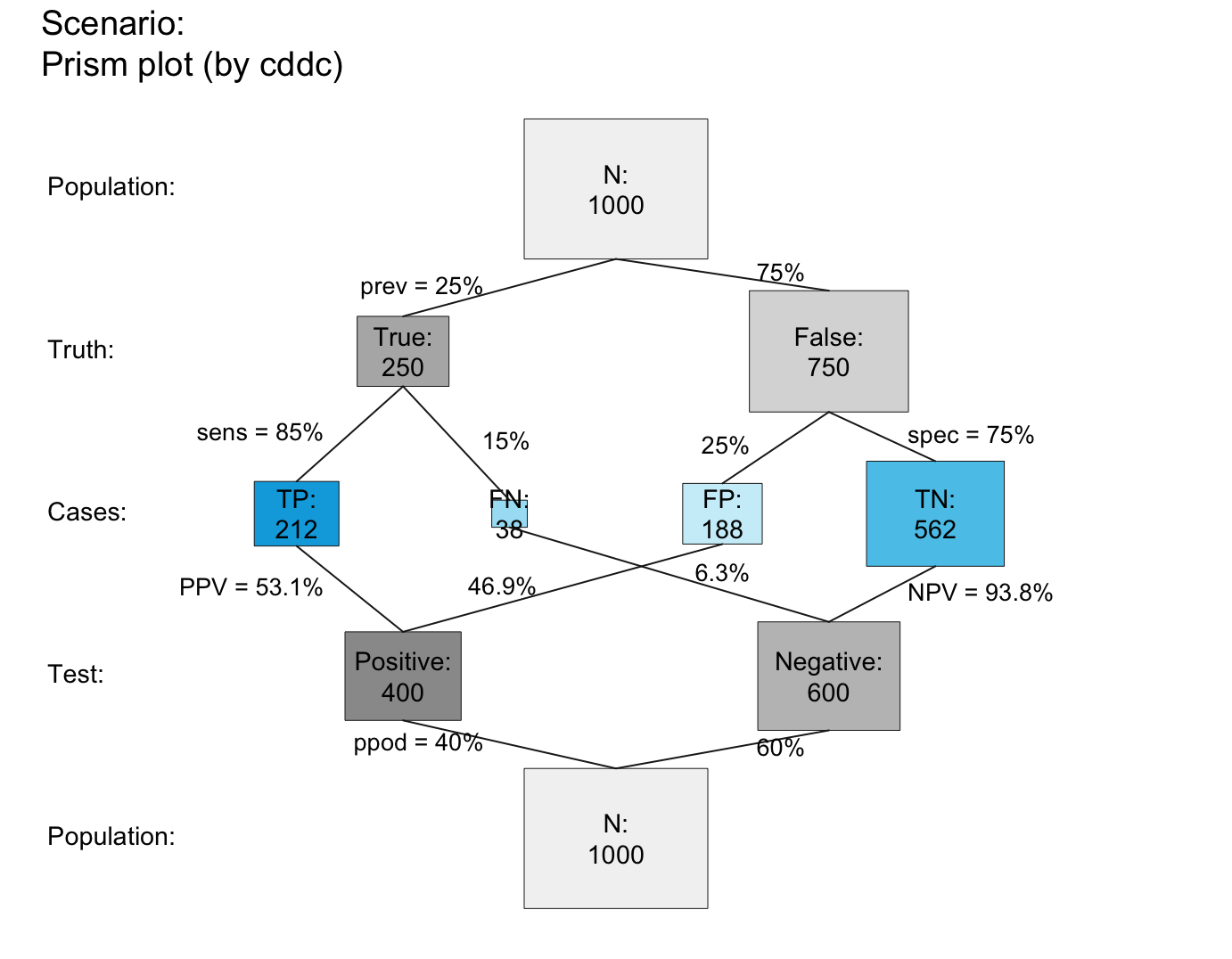plot_prism plots a network diagram of from a sufficient and valid set of 3 essential probabilities (prev, and sens or its complement mirt, and spec or its complement fart) or existing frequency information freq and a population size of N individuals.

plot_prism(prev = num$prev, sens = num$sens, mirt = NA,
spec = num$spec, fart = NA, N = num$N, by = "cddc",
area = "no", scale = "p", round = TRUE, f_lbl = "num",
f_lbl_sep = NA, f_lwd = 0, p_lwd = 1, p_scale = FALSE,
p_lbl = "mix", arr_c = NA, lbl_txt = txt,

Nothing (NULL).

## Details

plot_prism generalizes and replaces plot_fnet by removing the dependency on the R package diagram and providing many additional options.

plot_fnet for older (obsolete) version; plot_area for plotting mosaic plot (scaling area dimensions); plot_bar for plotting frequencies as vertical bars; plot_tab for plotting table (without scaling area dimensions); pal contains current color settings; txt contains current text settings.

Other visualization functions: plot.riskyr, plot_area, plot_bar, plot_curve, plot_fnet, plot_icons, plot_mosaic, plot_plane, plot_tab, plot_tree

## Examples

## Basics:
# (1) Using global prob and freq values:
plot_prism()  # default prism plot,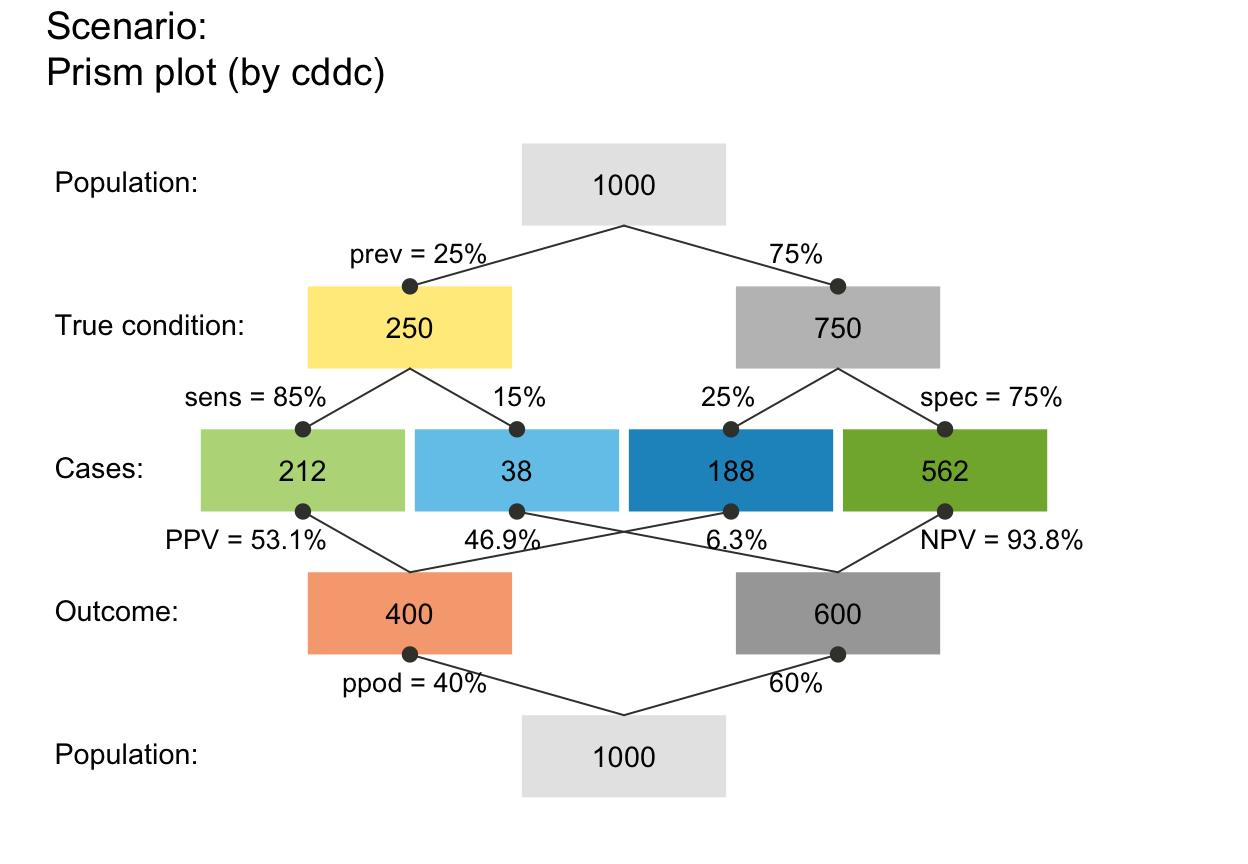# same as:
# plot_prism(by = "cddc", area = "no", scale = "p",
#            f_lbl = "num", f_lwd = 0, cex_lbl = .90,
#            p_lbl = "mix", arr_c = -2, cex_p_lbl = NA)

# (2) Providing values:
plot_prism(N = 10, prev = 1/2, sens = 4/5, spec = 3/5)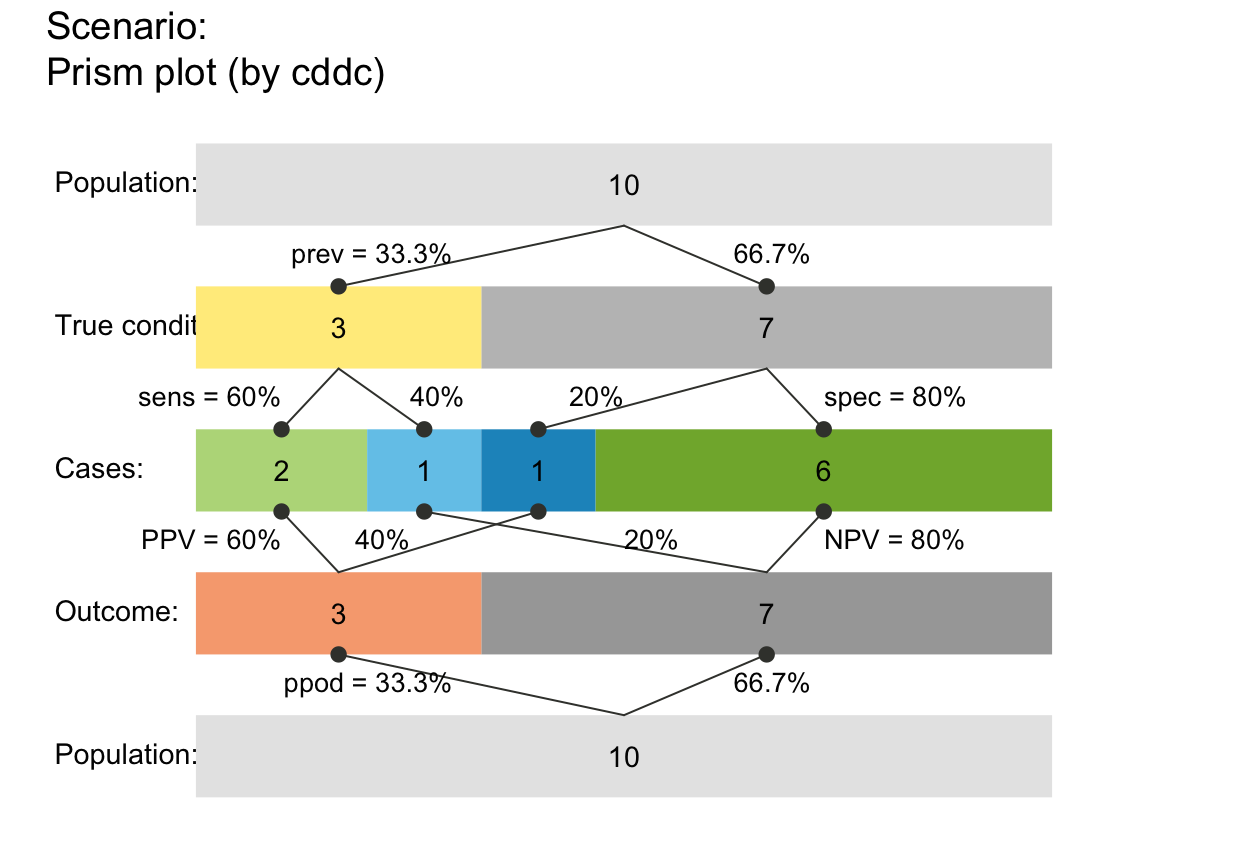plot_prism(N = 10, prev = 1/3, sens = 3/5, spec = 4/5, area = "hr")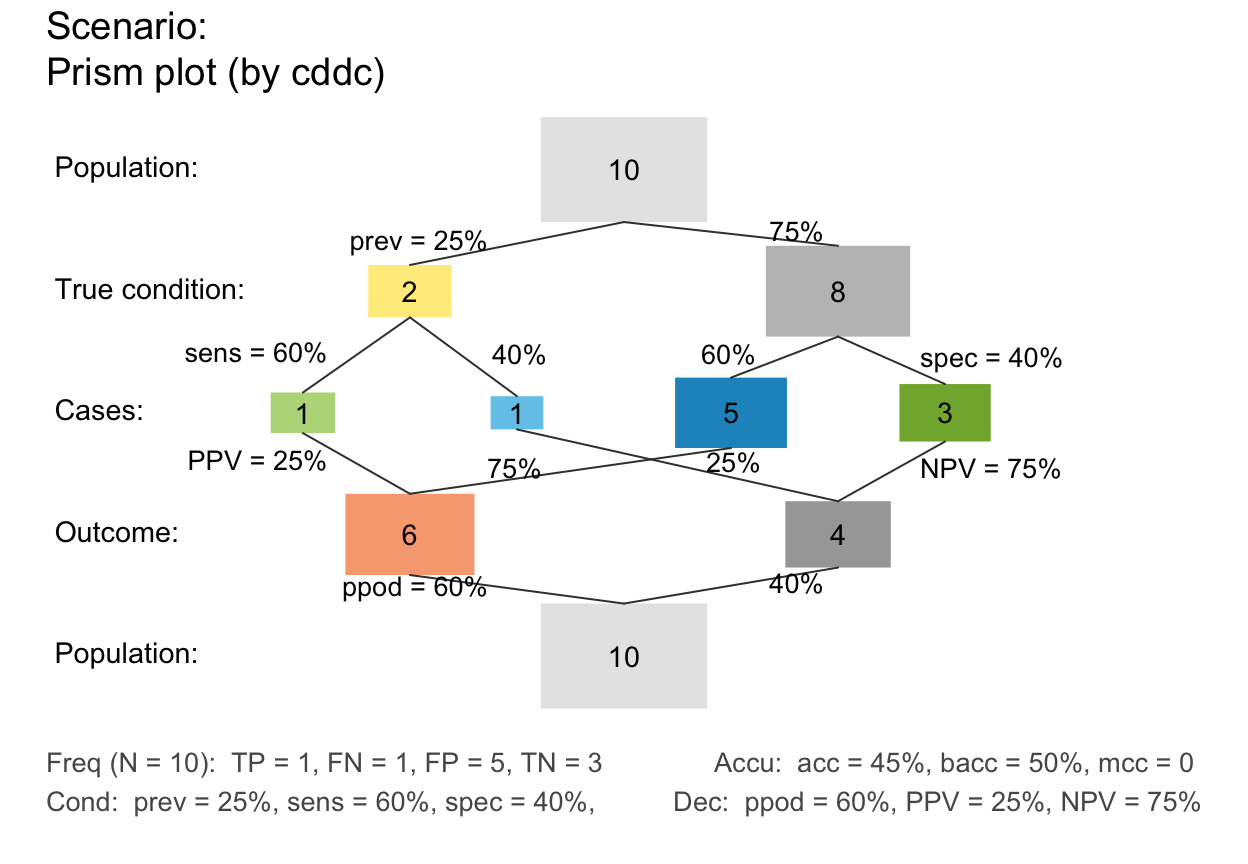plot_prism(N = 10, prev = 1/4, sens = 3/5, spec = 2/5, area = "sq", mar_notes = TRUE)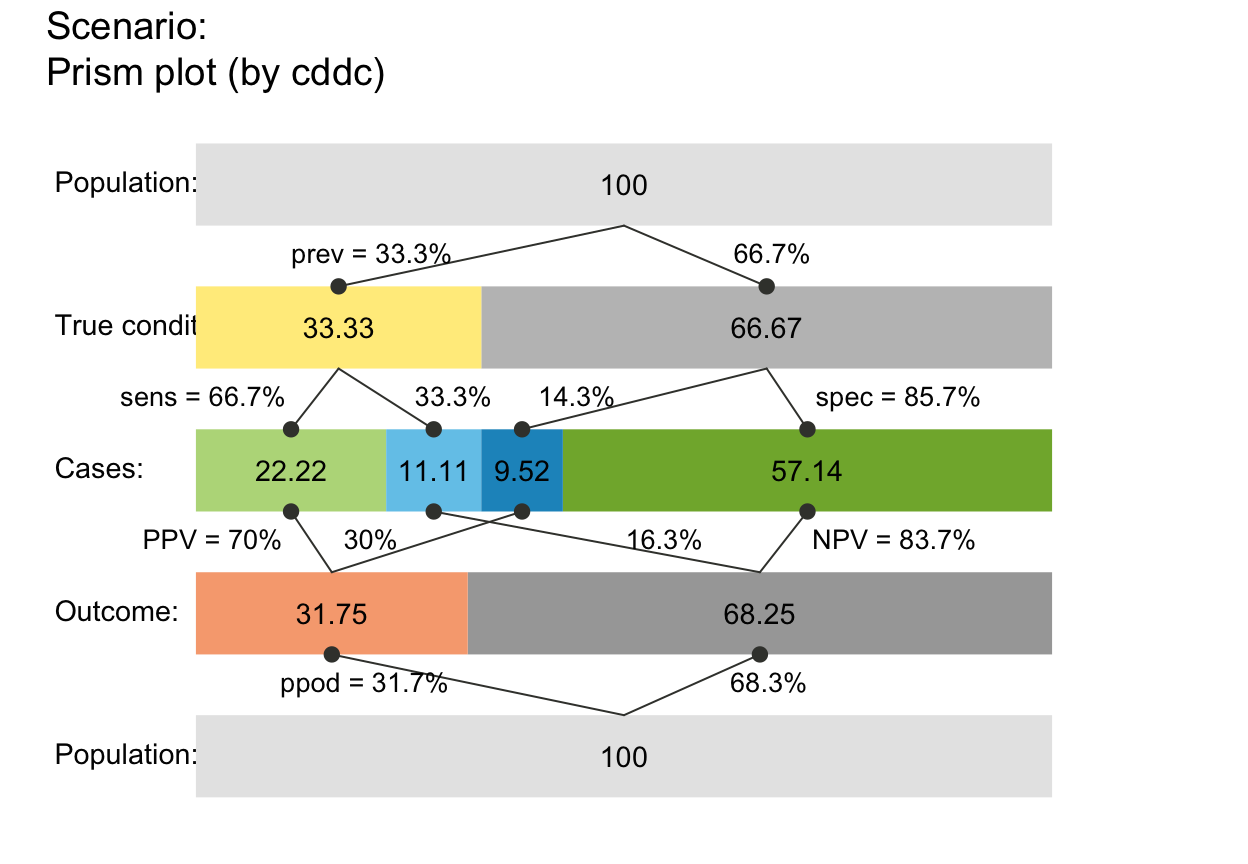## Custom color and text settings:
plot_prism(col_pal = pal_bw, f_lwd = .5, p_lwd = .5, lty = 2, # custom fbox color, prob links,
font = 3, cex_p_lbl = .75)                         # and text labels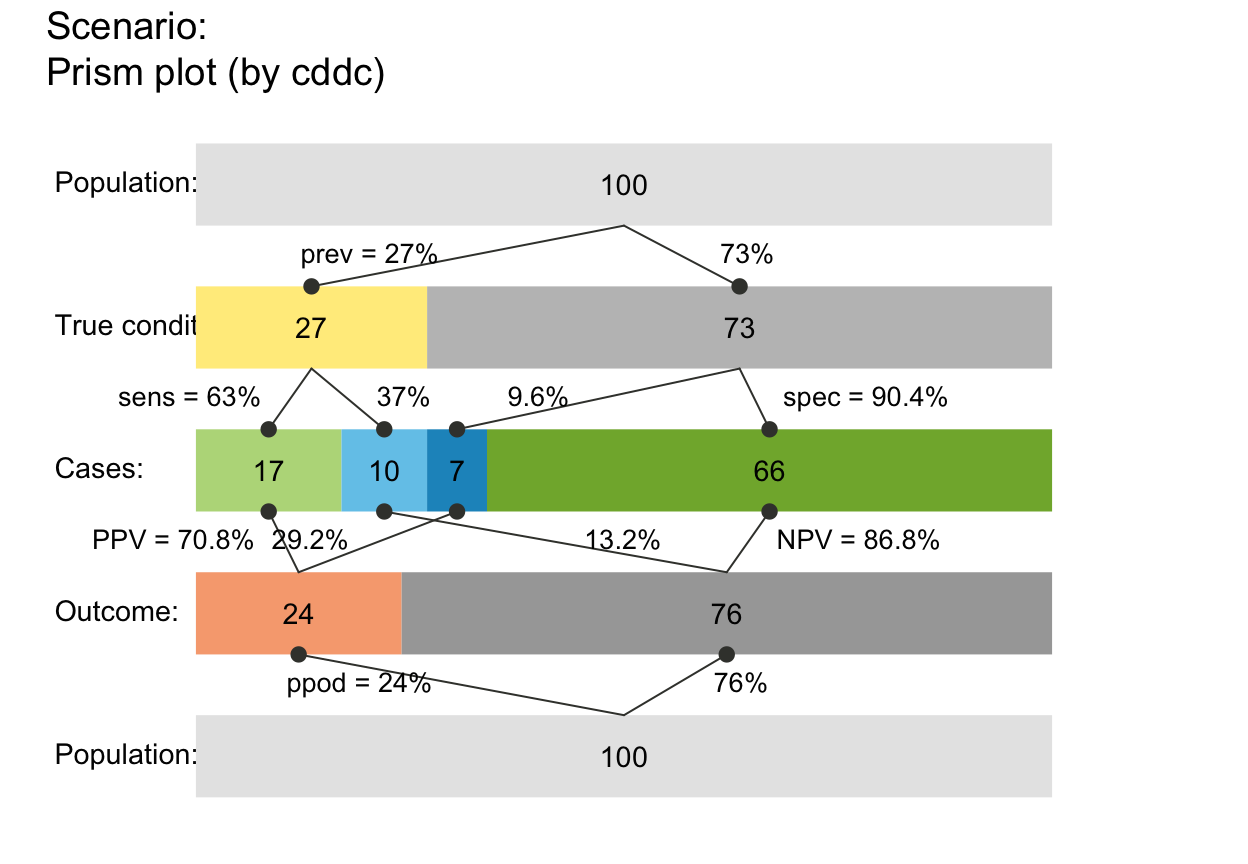my_txt <- init_txt(cond_lbl = "The Truth", cond_true_lbl = "so true", cond_false_lbl = "so false",
hi_lbl = "TP", mi_lbl = "FN", fa_lbl = "FP", cr_lbl = "TN")
my_col <- init_pal(N_col = rgb(0, 169, 224, max = 255),  # seeblau
hi_col = "gold", mi_col = "firebrick1", fa_col = "firebrick2", cr_col = "orange")
plot_prism(f_lbl = "nam", lbl_txt = my_txt,
col_pal = my_col, f_lwd = .5)## Local values and custom color/txt settings:
plot_prism(N = 7, prev = 1/2, sens = 3/5, spec = 4/5, round = FALSE,
by = "cdac", lbl_txt = txt_org, f_lbl = "namnum", f_lbl_sep = ":\n",
f_lwd = 1, col_pal = pal_rgb)  # custom colors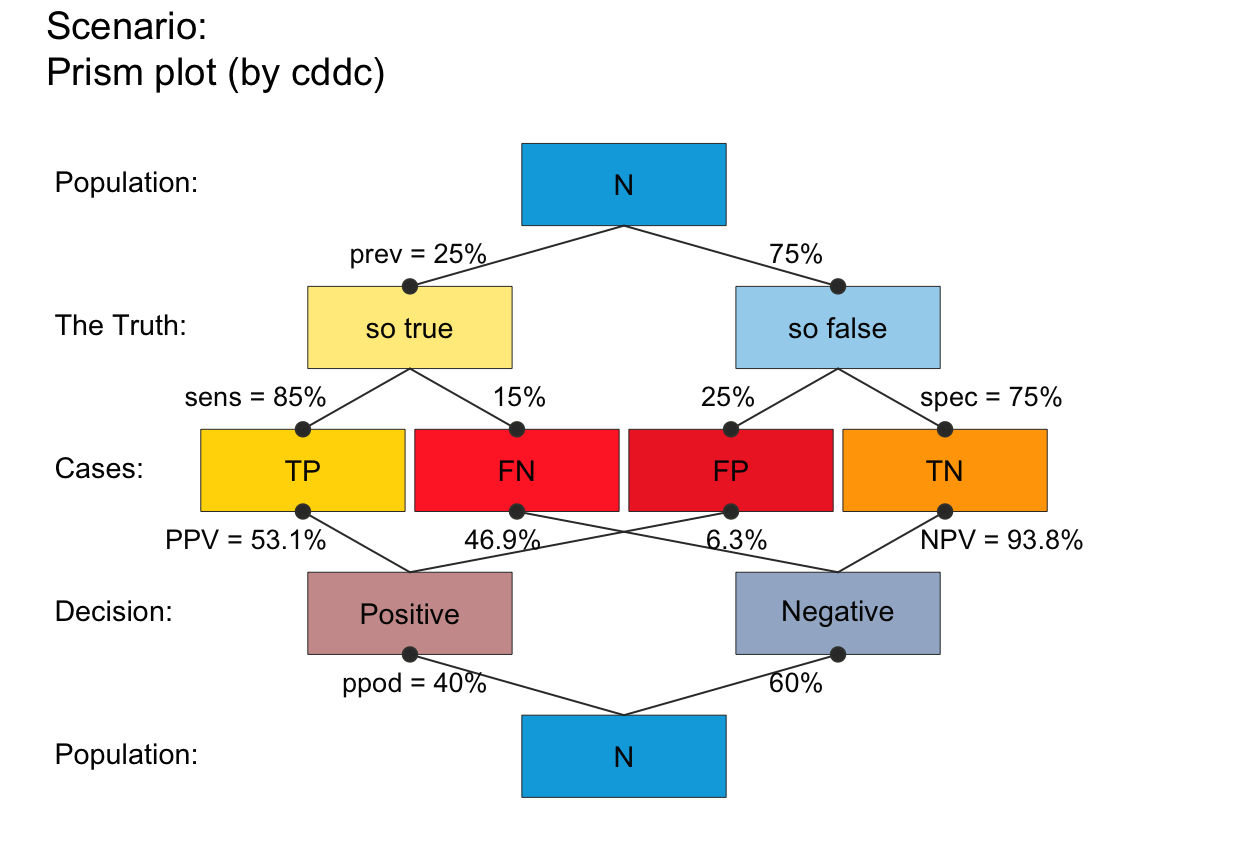plot_prism(N = 5, prev = 1/2, sens = .8, spec = .5, scale = "p",  # note scale!
by = "cddc", area = "hr", col_pal = pal_bw, f_lwd = 1) # custom colors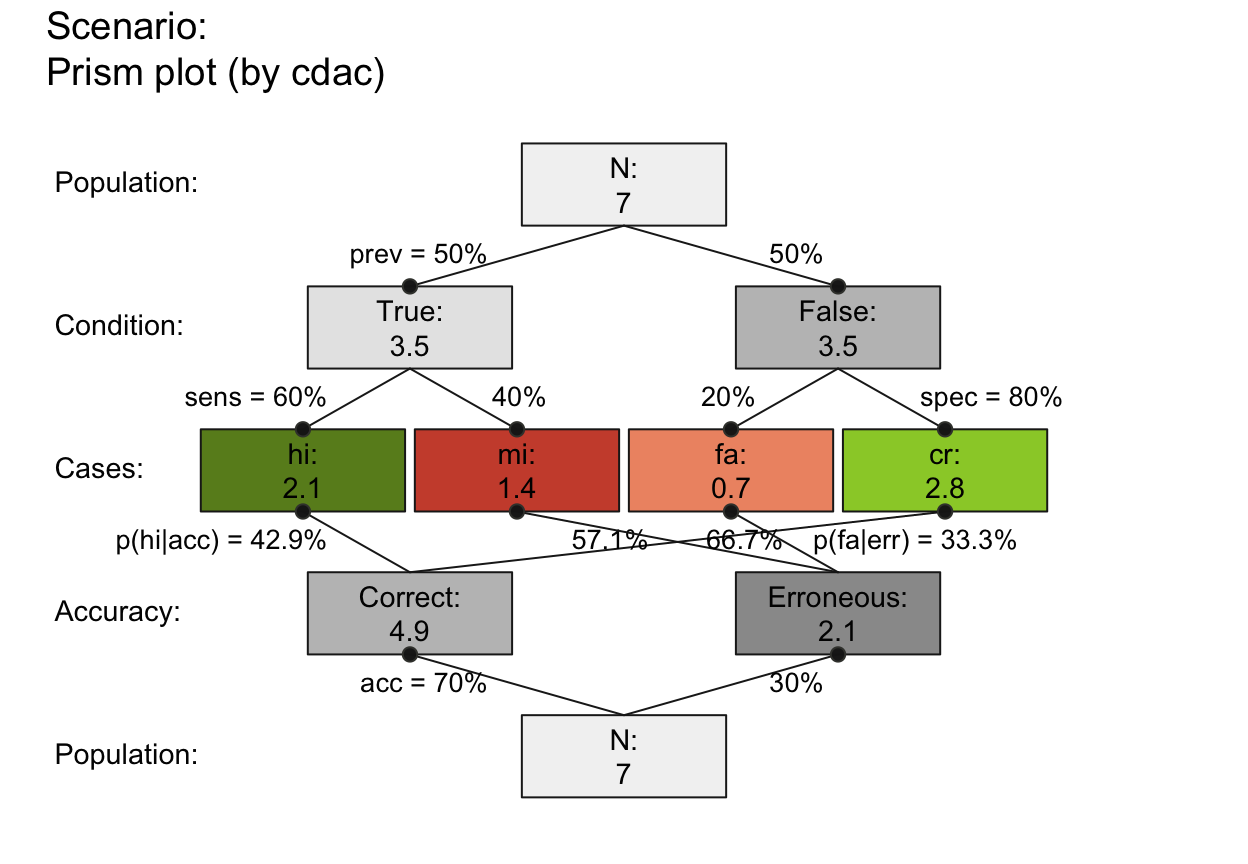plot_prism(N = 3, prev = .50, sens = .50, spec = .50, scale = "p",              # note scale!
area = "sq", lbl_txt = txt_org, f_lbl = "namnum", f_lbl_sep = ":\n", # custom text
col_pal = pal_kn, f_lwd = .5)                                        # custom colors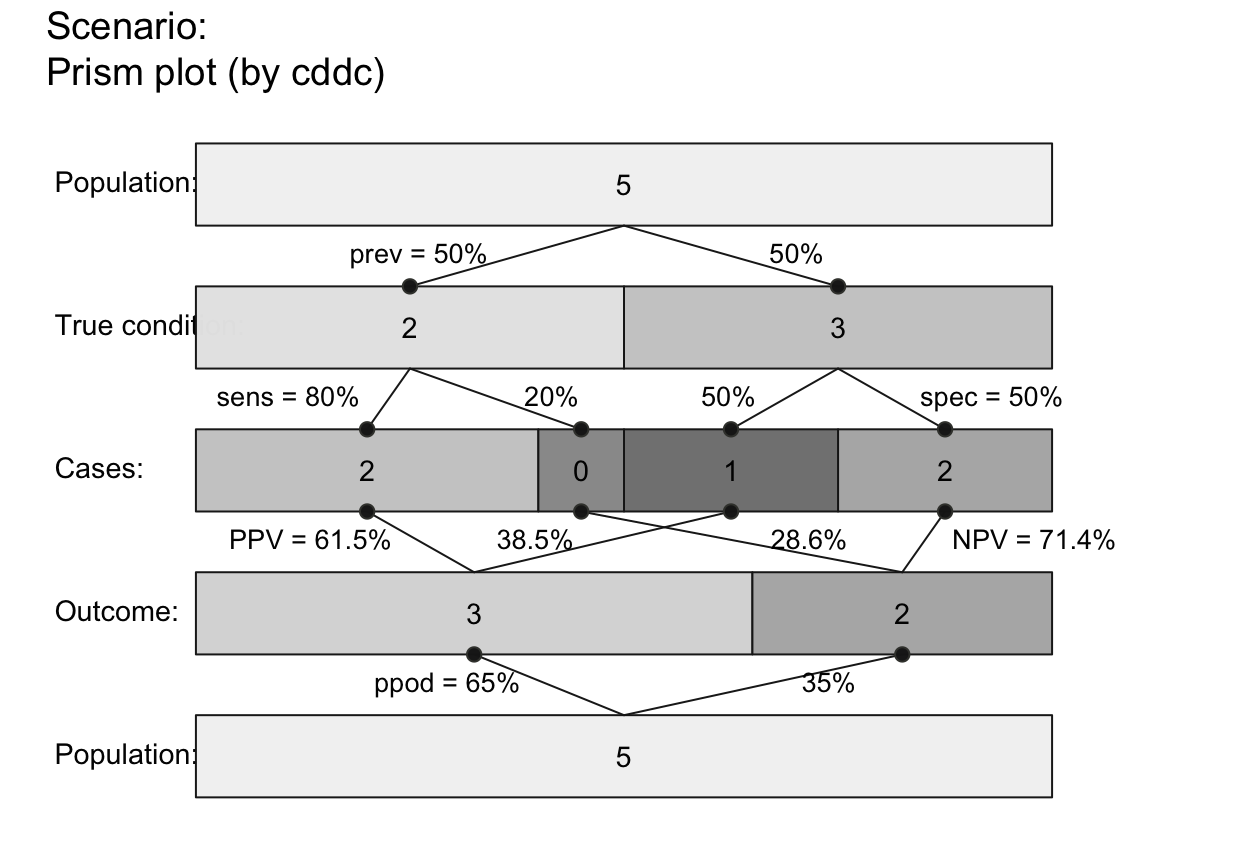## Plot versions:
# (A) tree/single tree (nchar(by) == 2):
#     3 versions:
plot_prism(by = "cd", f_lbl = "def", col_pal = pal_mod) # by condition (freq boxes: hi mi fa cr)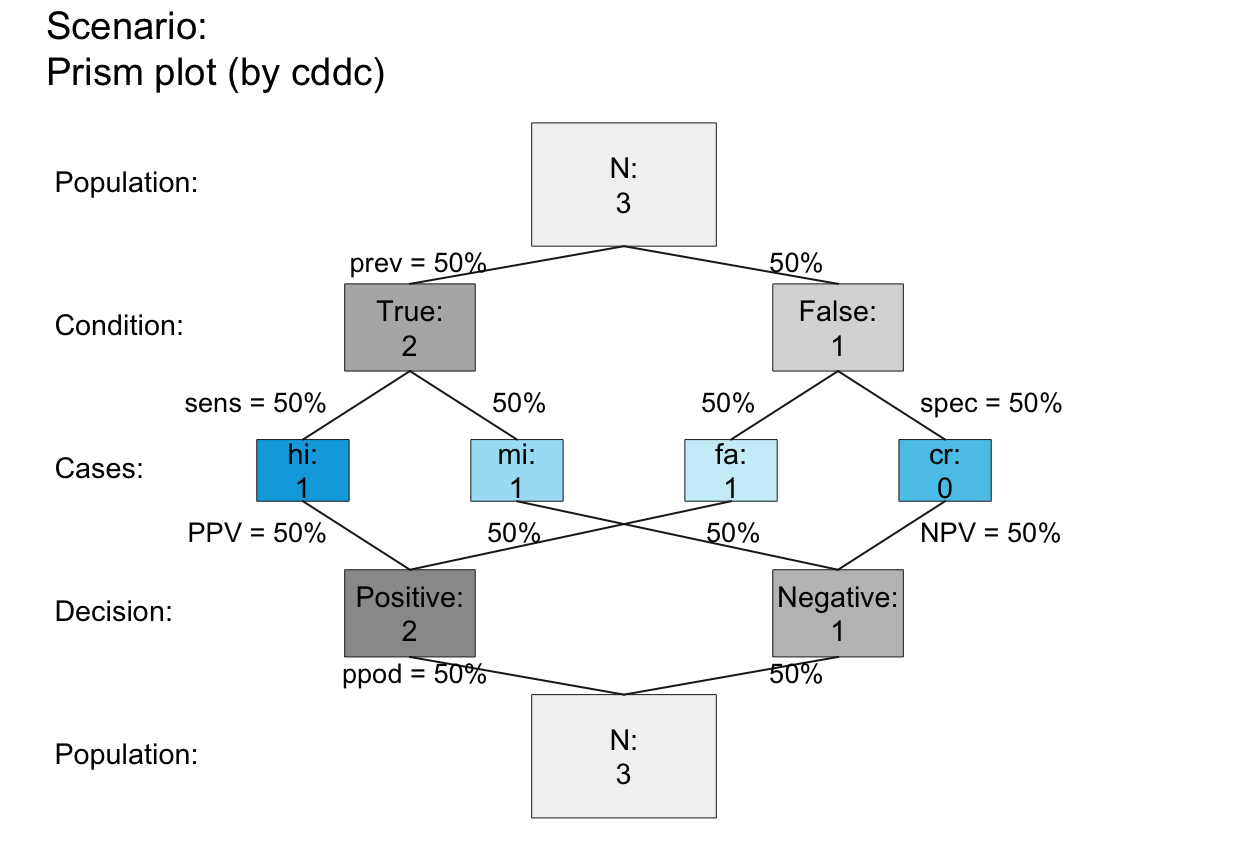plot_prism(by = "dc", f_lbl = "def", col_pal = pal_mod) # by decision  (freq boxes: hi fa mi cr)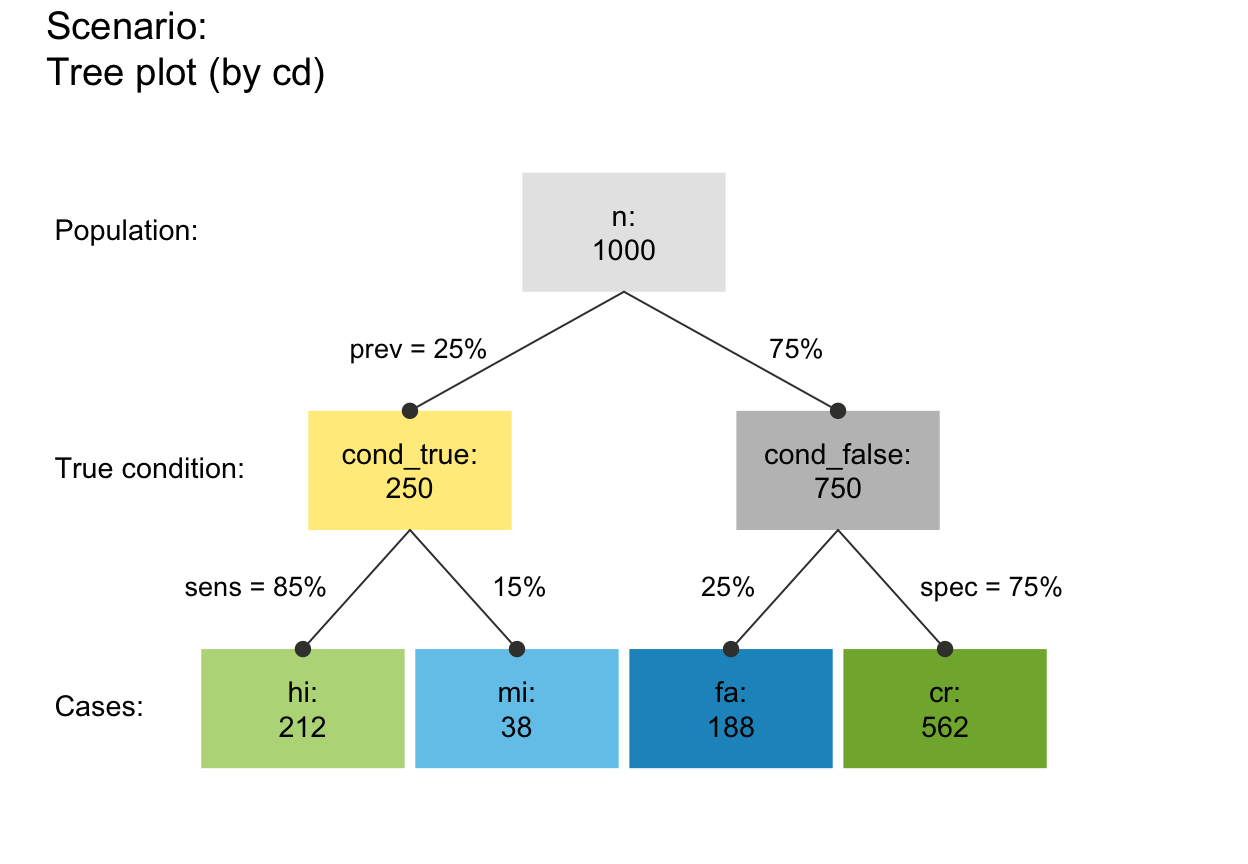plot_prism(by = "ac", f_lbl = "def", col_pal = pal_mod) # by decision  (freq boxes: hi cr mi fa)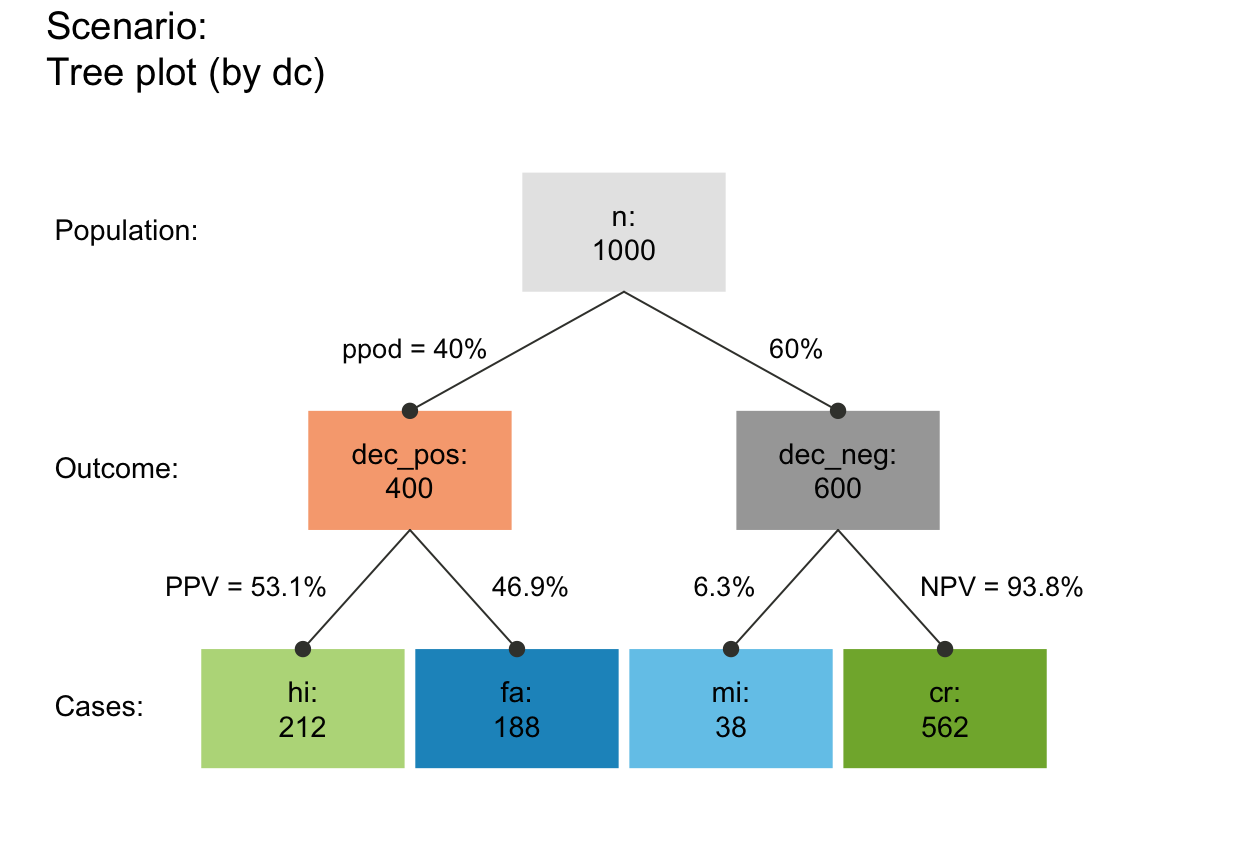# (B) prism/double tree (nchar(by) == 4):
#     6 (3 x 2) versions (+ 3 redundant ones):
plot_prism(by = "cddc")  # v01 (default)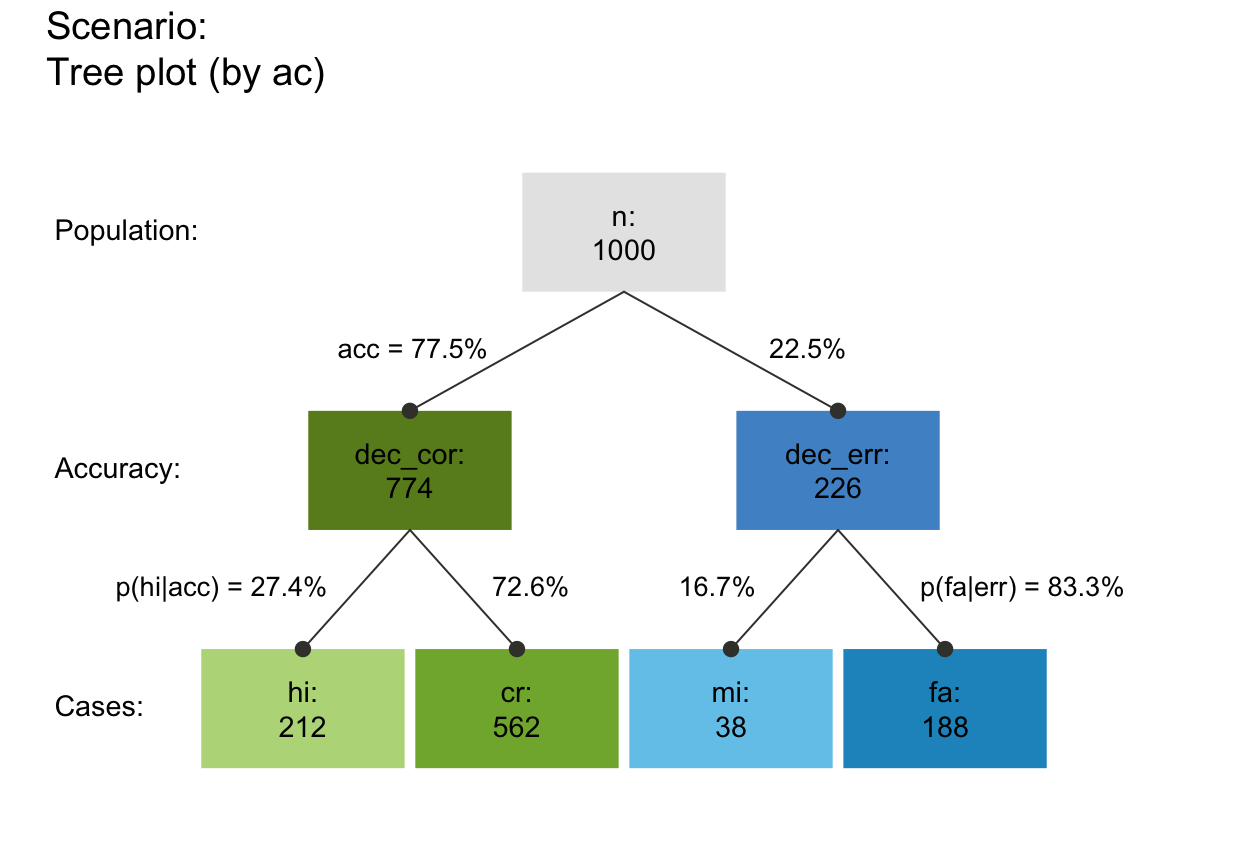plot_prism(by = "cdac")  # v02plot_prism(by = "cdcd")  # (+) Message#> Specified 2 identical perspectives.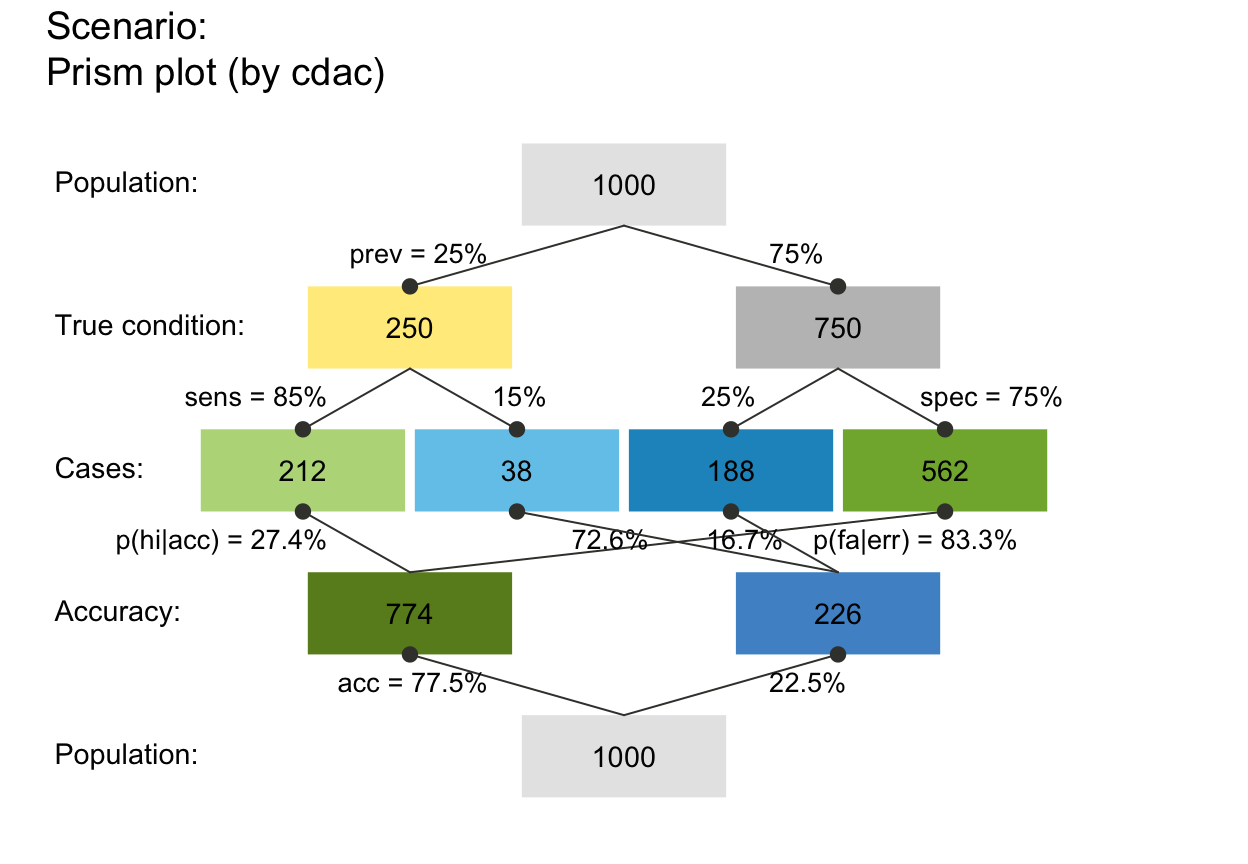plot_prism(by = "dccd")  # v03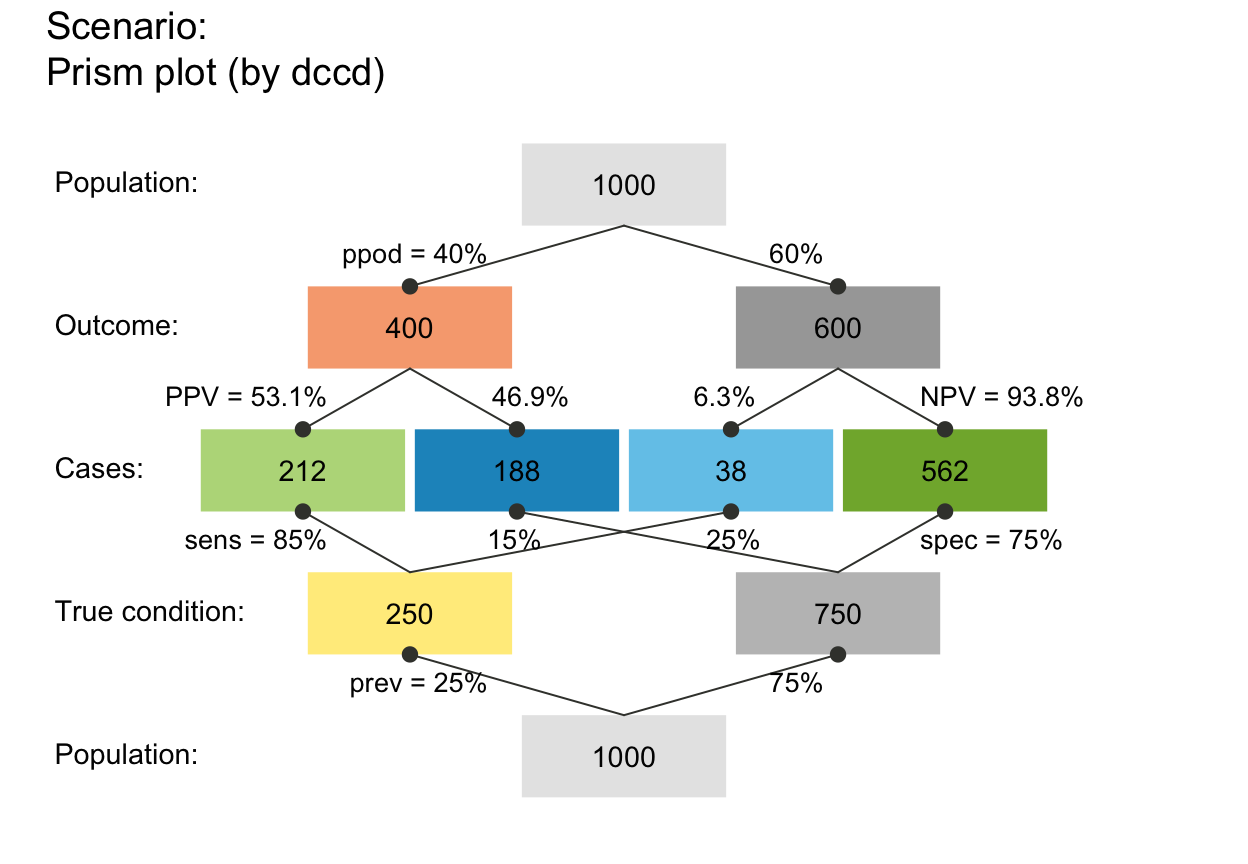plot_prism(by = "dcac")  # v04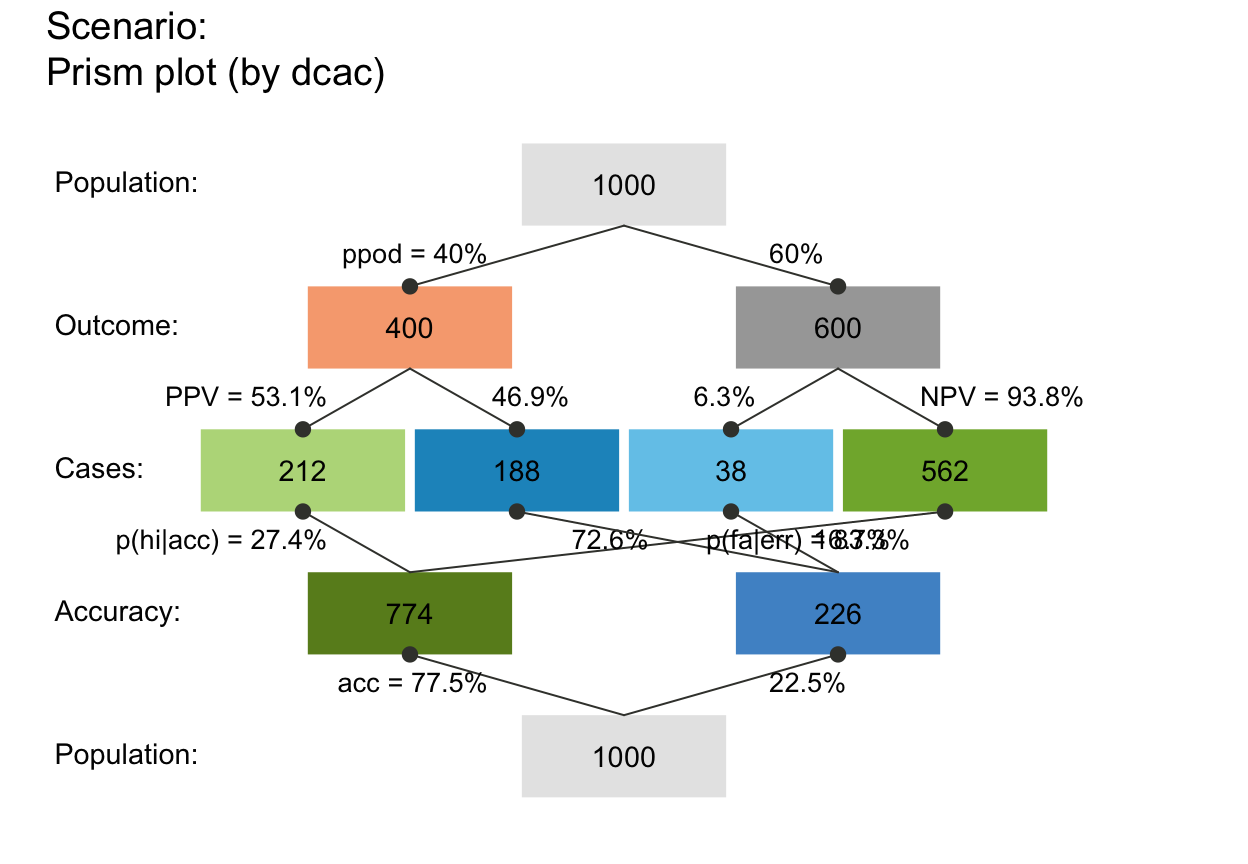plot_prism(by = "dcdc")  # (+) Message#> Specified 2 identical perspectives.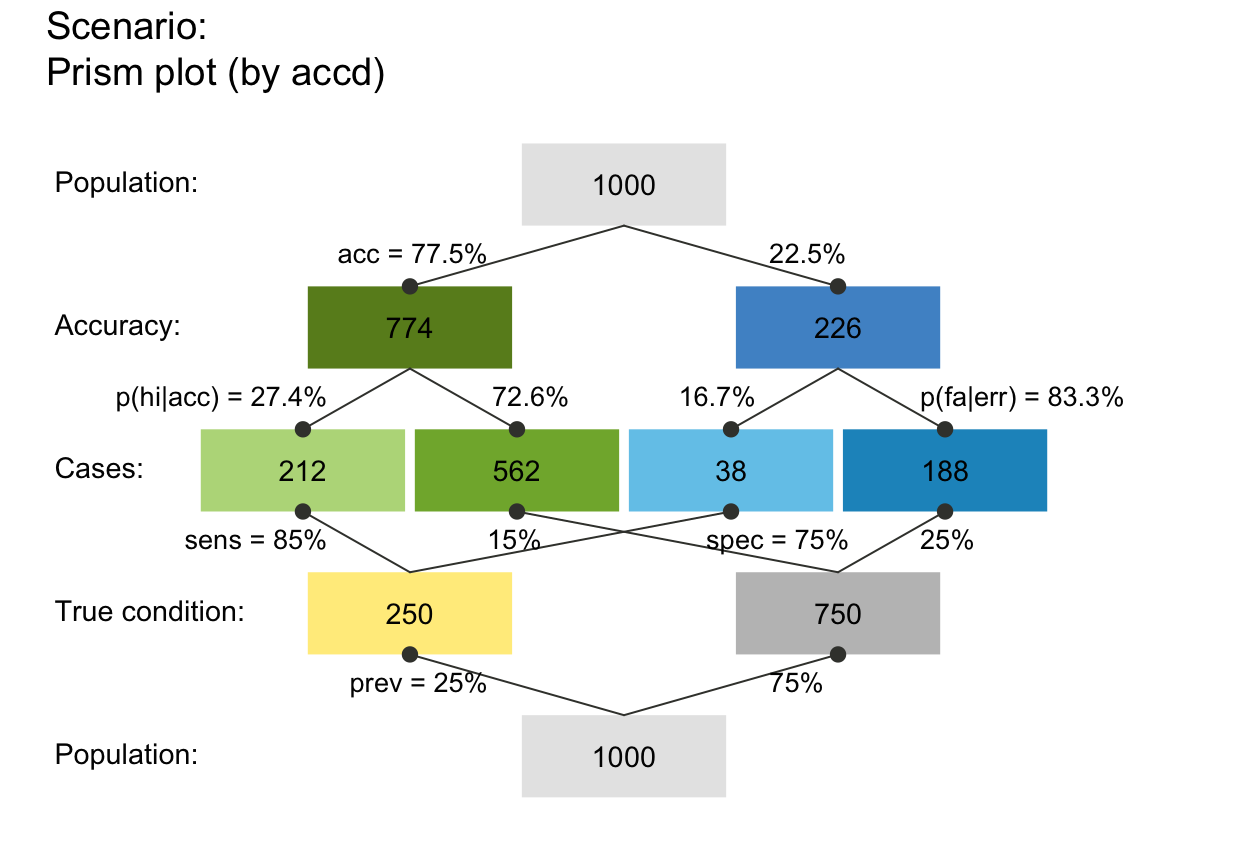plot_prism(by = "accd")  # v05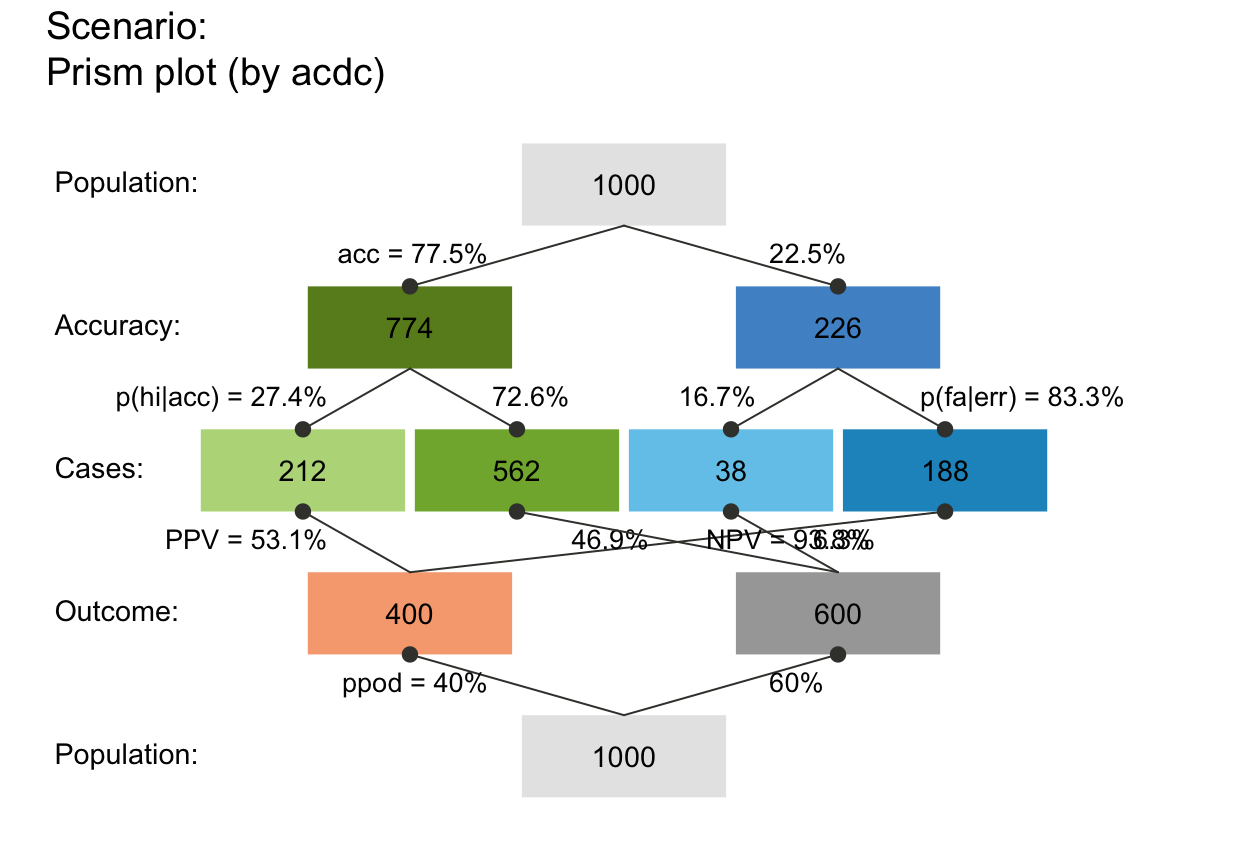plot_prism(by = "acdc")  # v06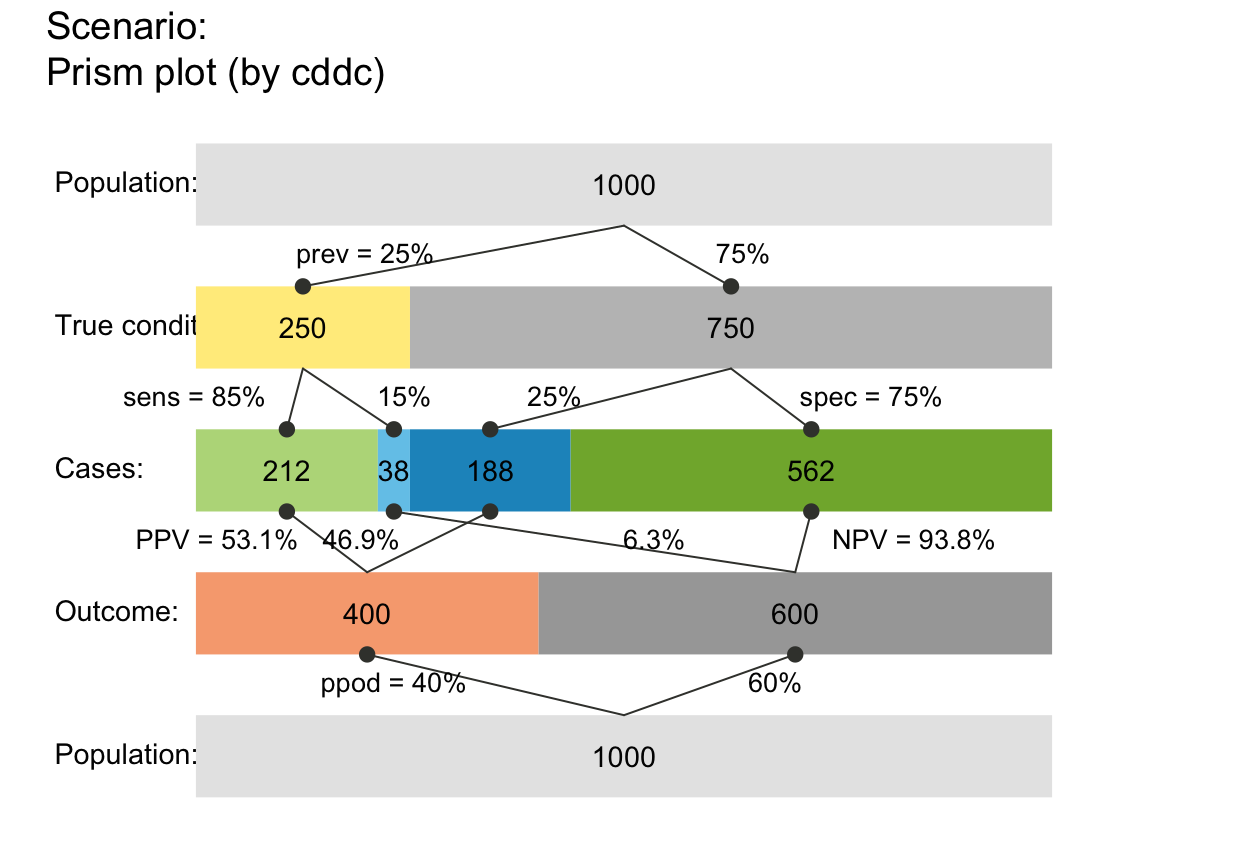plot_prism(by = "acac")  # (+) Message#> Specified 2 identical perspectives.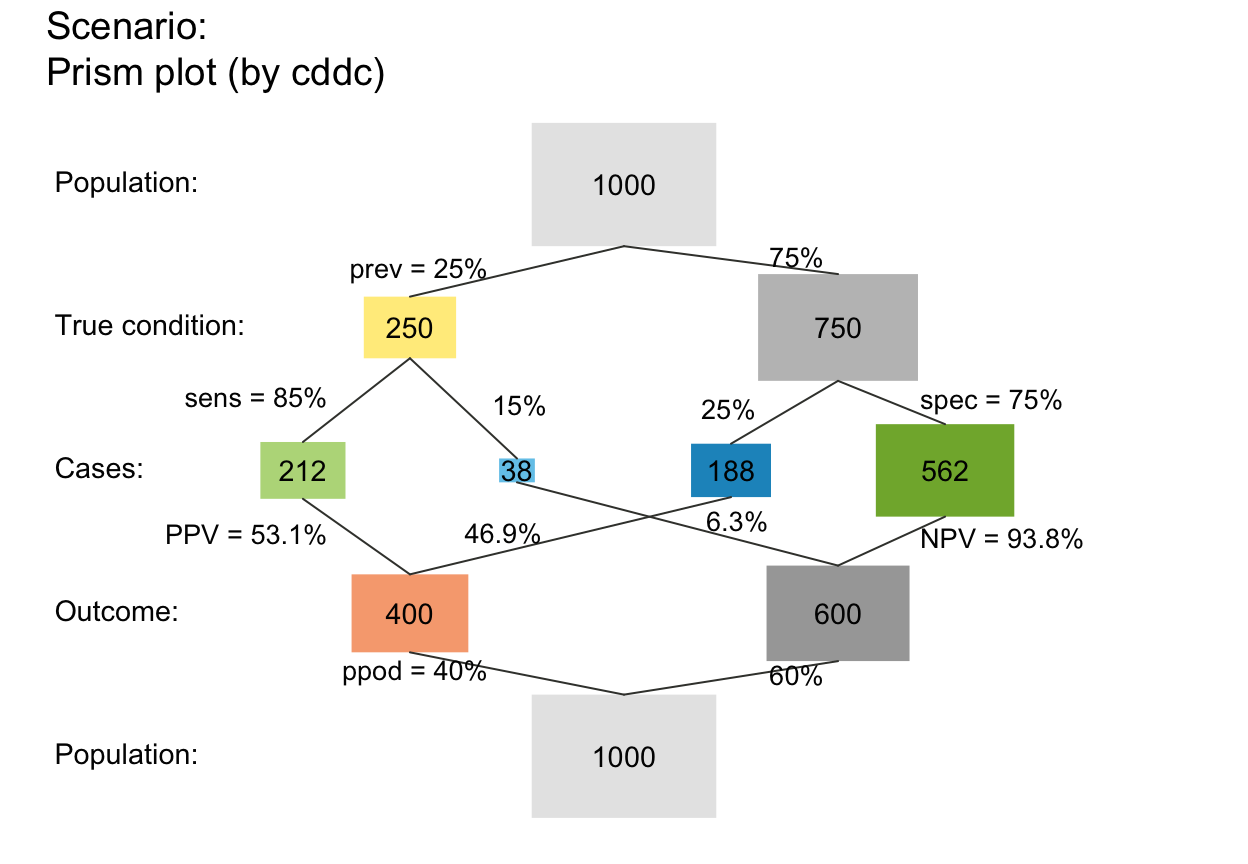## Other options:

# area:
plot_prism(area = "no")  # rectangular boxes (default): (same if area = NA/NULL)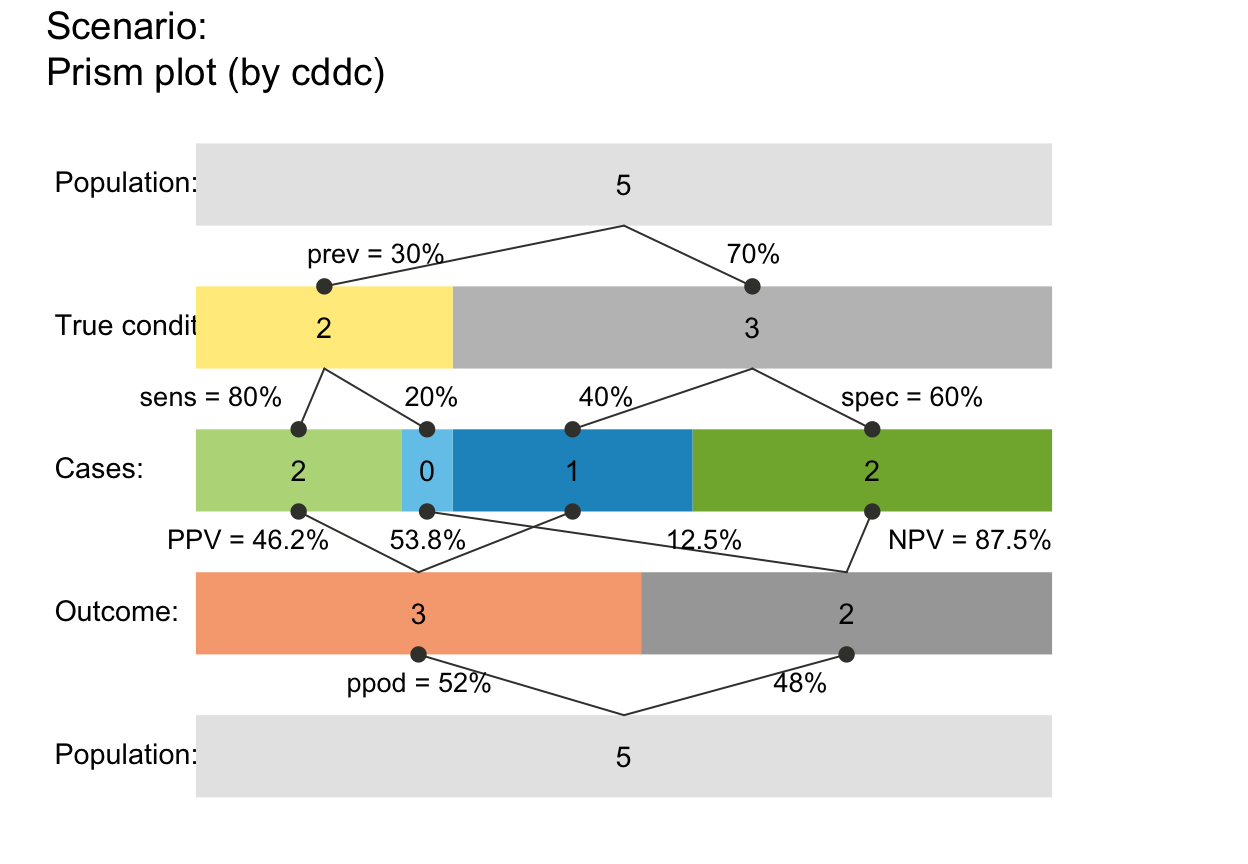plot_prism(area = "hr")  # horizontal rectangles (widths on each level sum to N)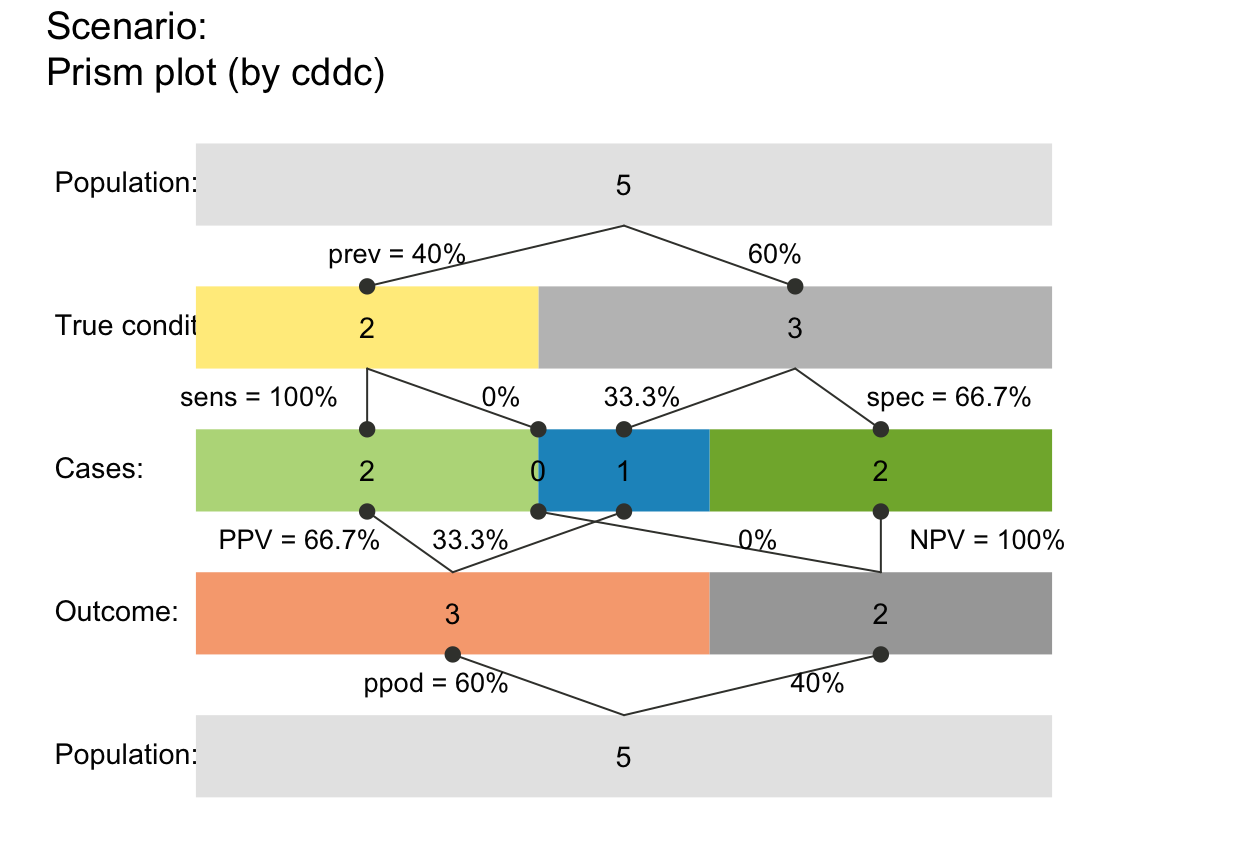plot_prism(area = "sq")  # squares (areas on each level sum to N)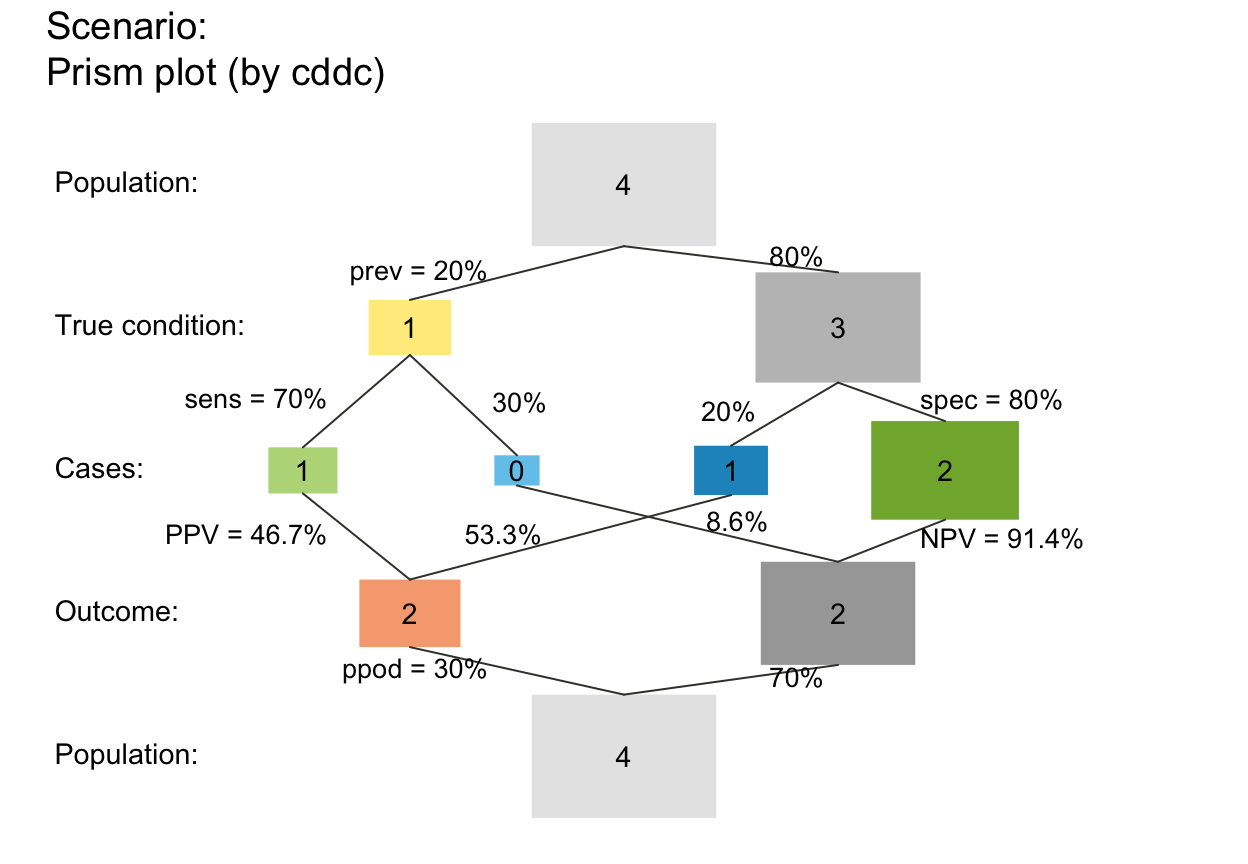# scale (matters for scaled areas and small N):
plot_prism(N = 5, prev = .3, sens = .8, spec = .6,
area = "hr", scale = "p")  # widths scaled by prob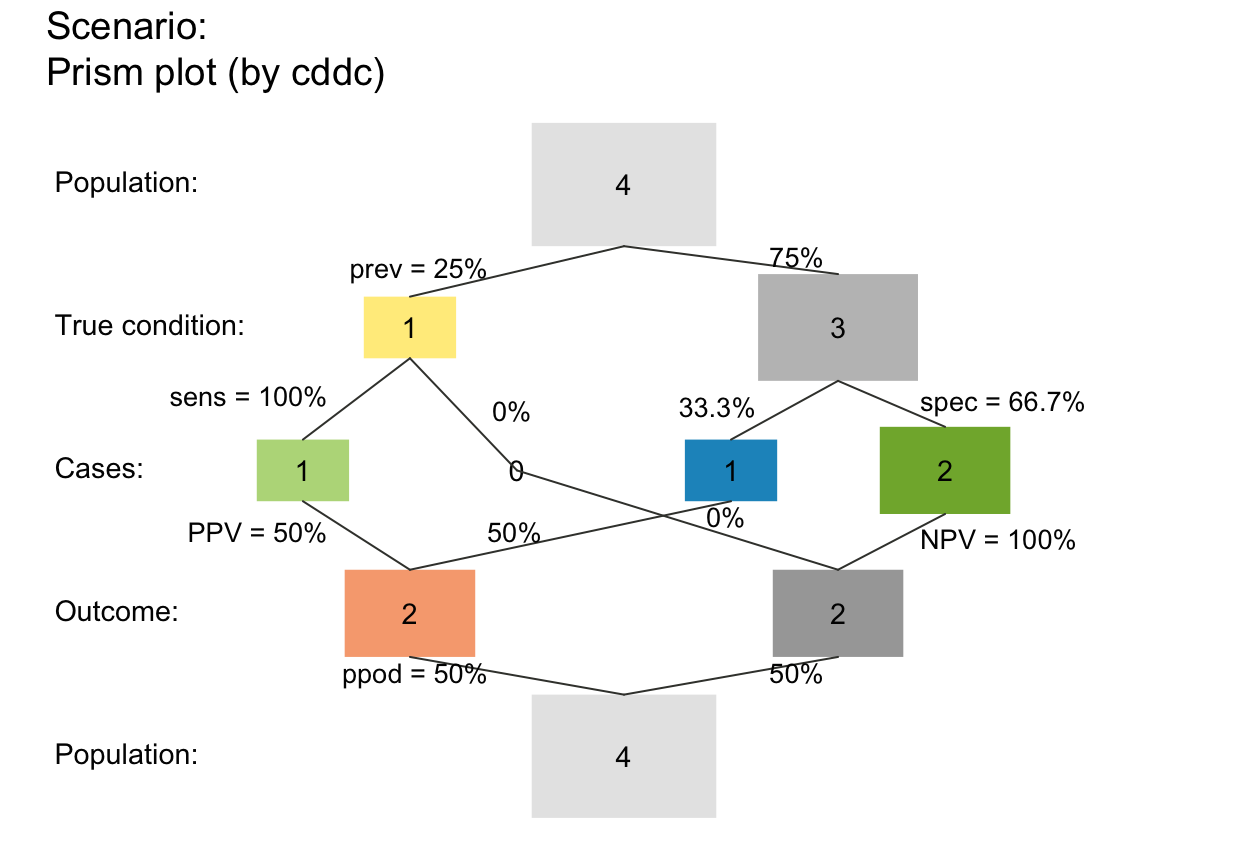plot_prism(N = 5, prev = .3, sens = .8, spec = .6,
area = "hr", scale = "f")  # widths scaled by (rounded or non-rounded) freq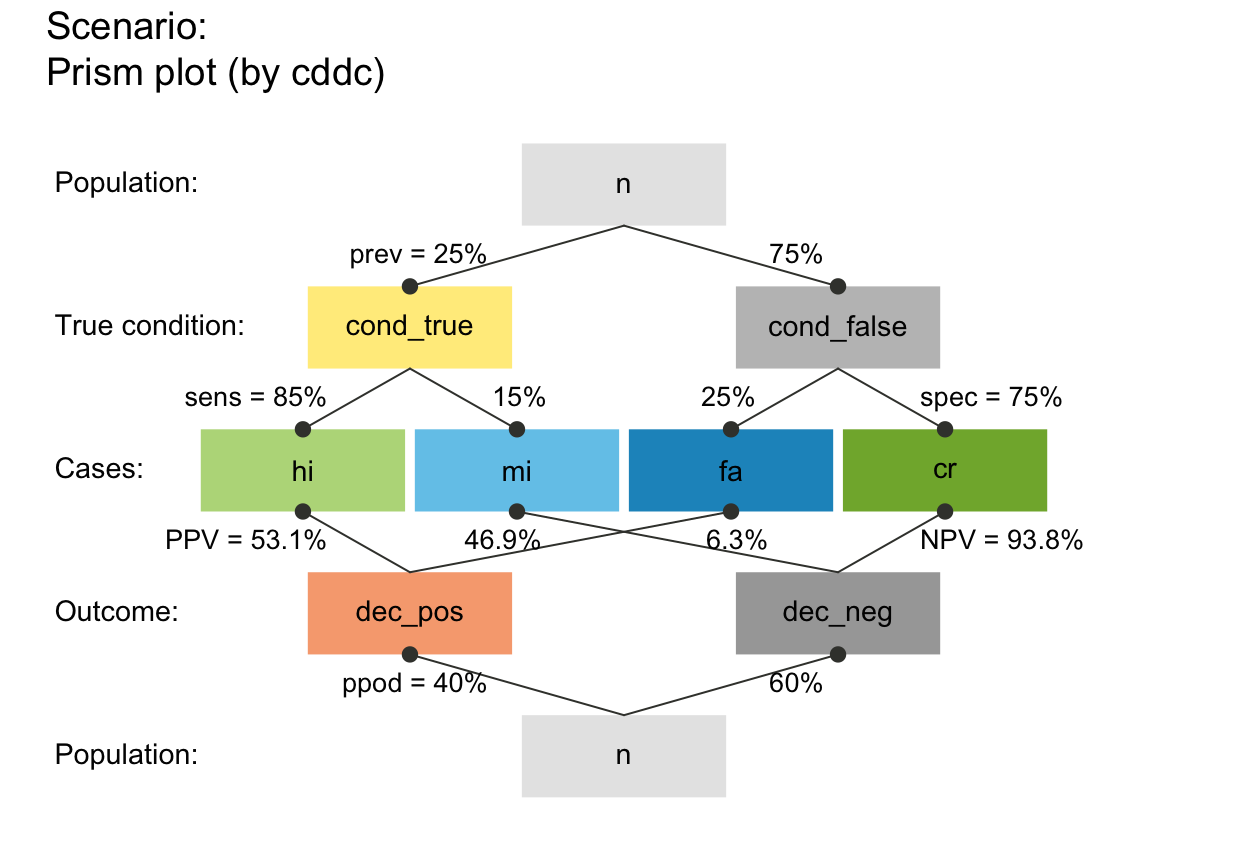plot_prism(N = 4, prev = .2, sens = .7, spec = .8,
area = "sq", scale = "p")  # areas scaled by prob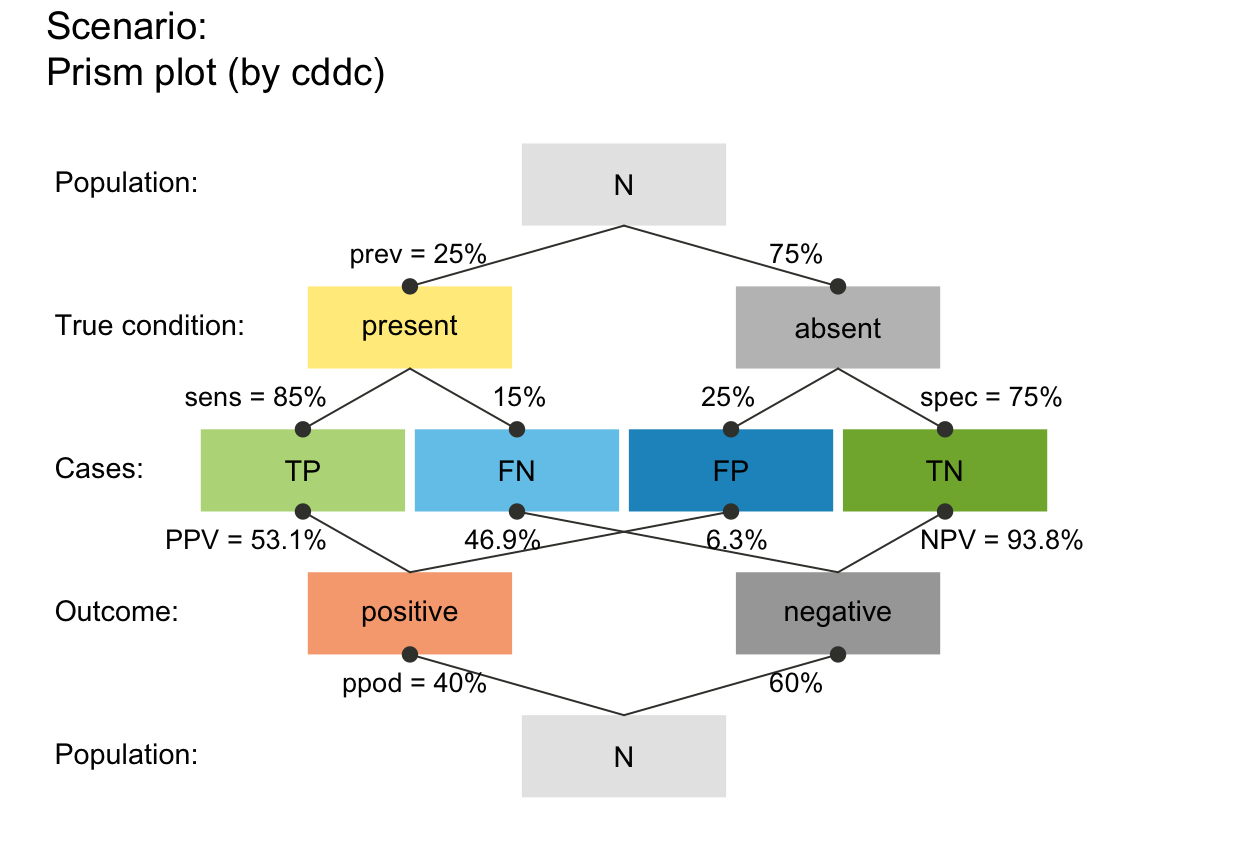plot_prism(N = 4, prev = .2, sens = .7, spec = .8,
area = "sq", scale = "f")  # areas scaled by (rounded or non-rounded) freq## Frequency boxes:

# f_lbl:
plot_prism(f_lbl = "abb")     # abbreviated freq names (variable names)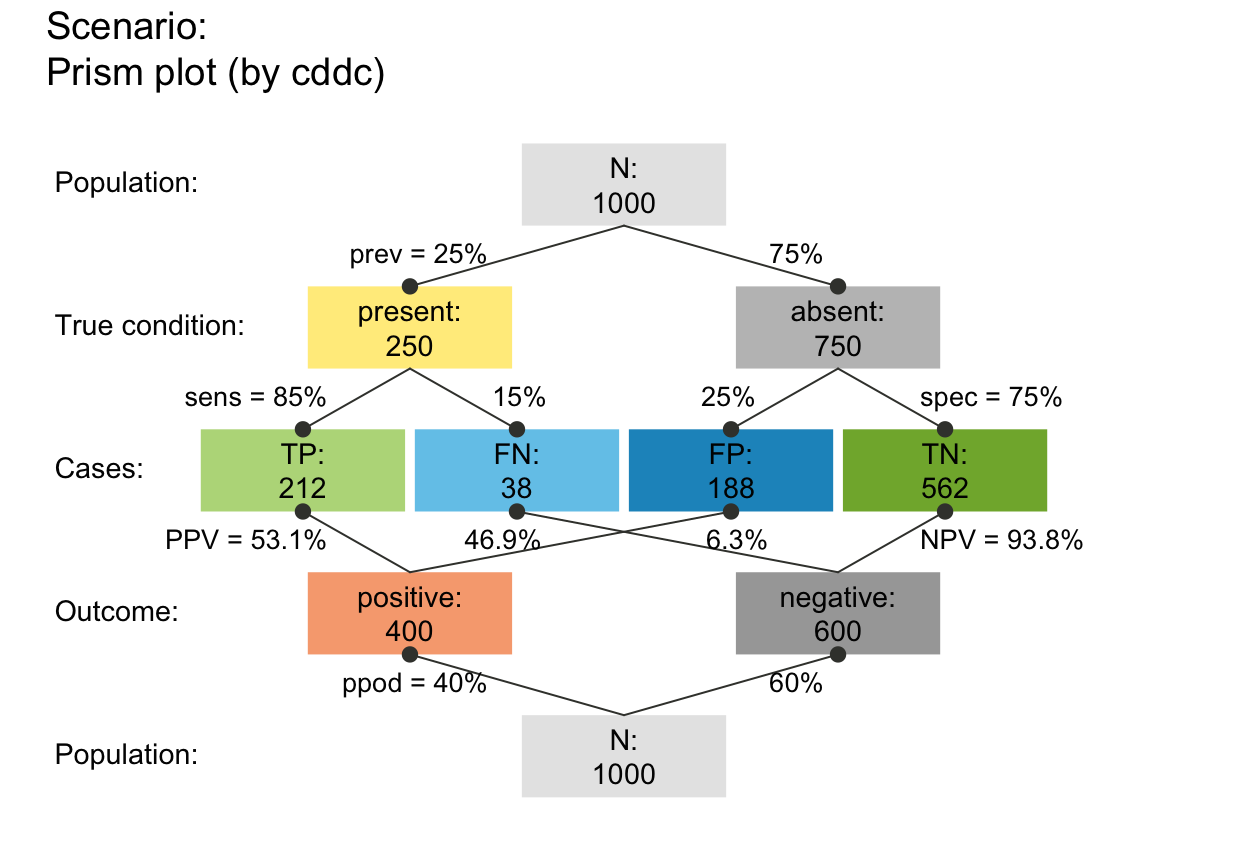plot_prism(f_lbl = "nam")     # only freq names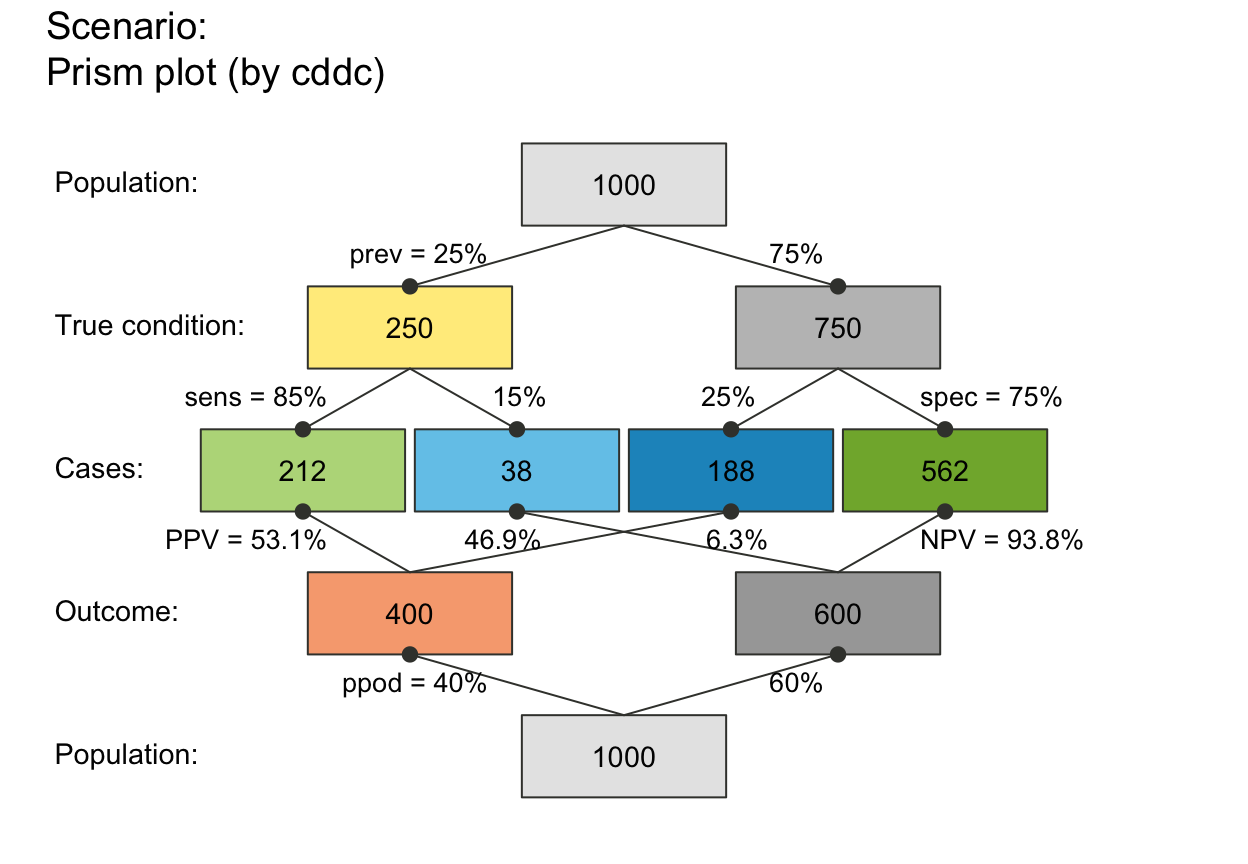plot_prism(f_lbl = "num")     # only numeric freq values (default)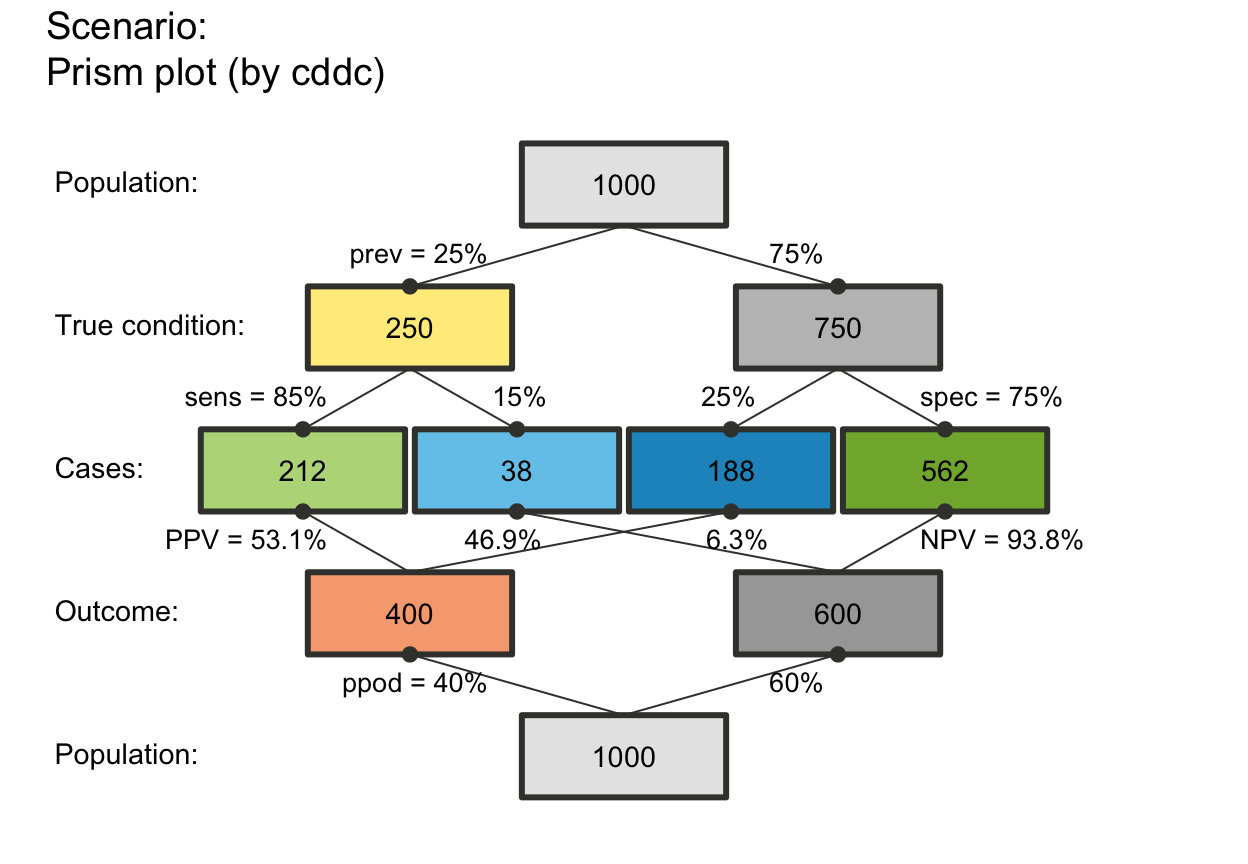plot_prism(f_lbl = "namnum")  # names and numeric freq values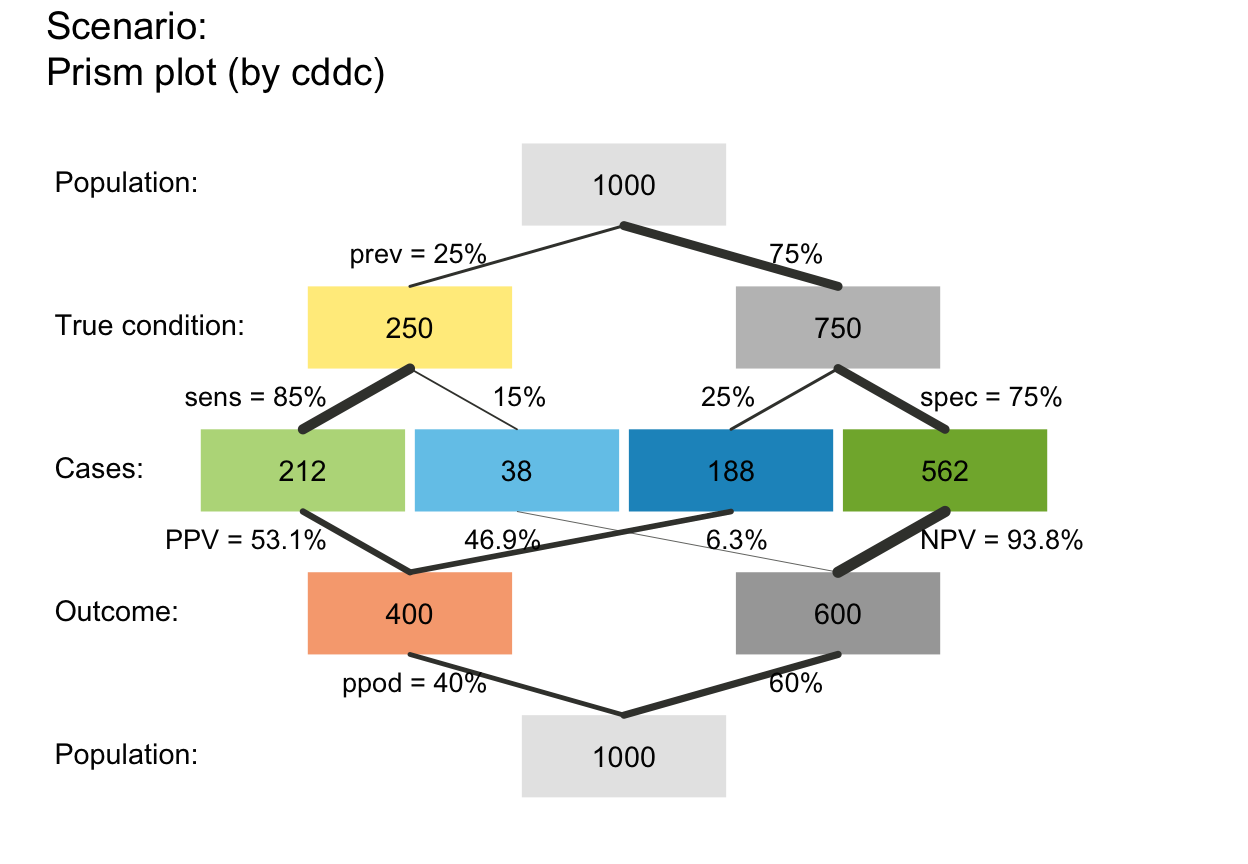plot_prism(f_lbl = "namnum", cex_lbl = .75)  # smaller freq labelsplot_prism(f_lbl = NA)        # no freq labelsplot_prism(f_lbl = "def")     # informative default: short name and numeric value (abb = num)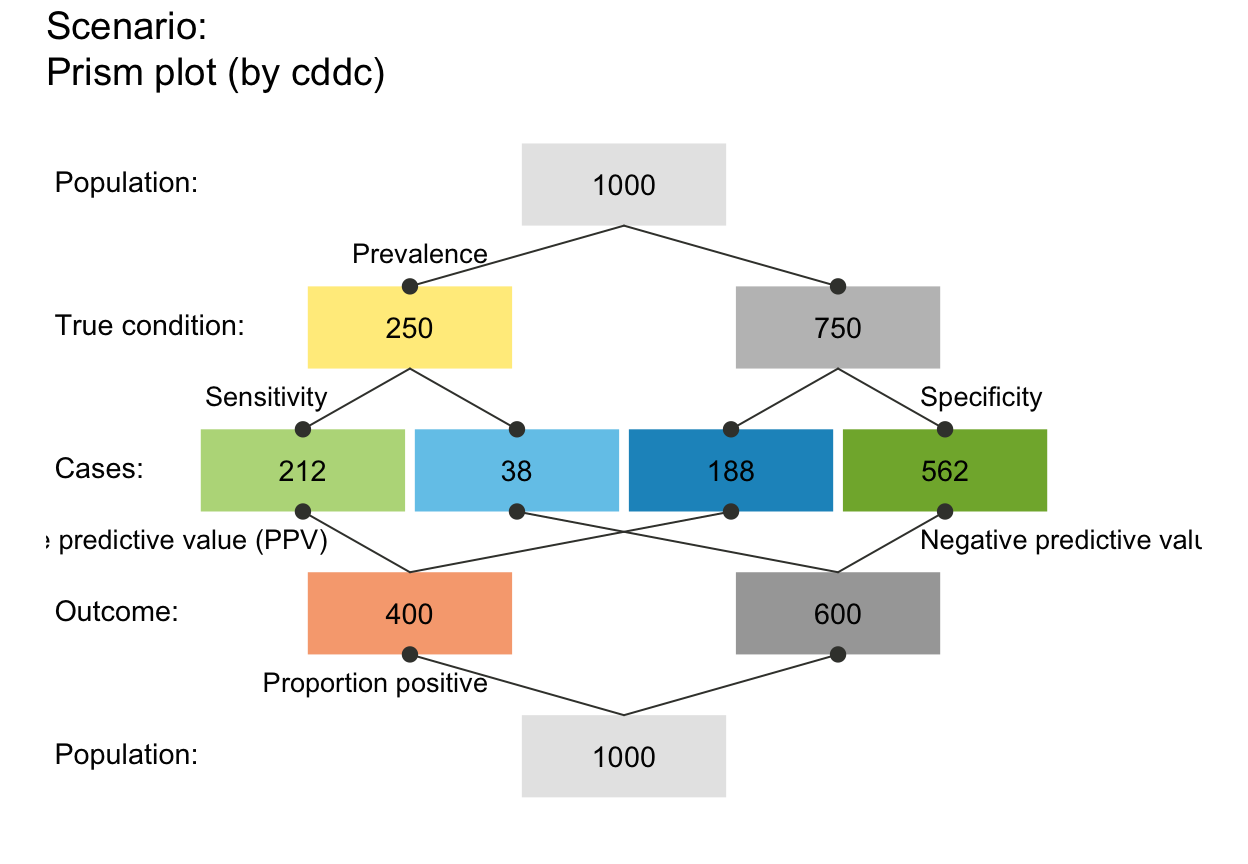# f_lwd:
plot_prism(f_lwd =  0)  # no lines (default), set to tiny_lwd = .001, lty = 0 (same if NA/NULL)plot_prism(f_lwd =  1)  # basic lines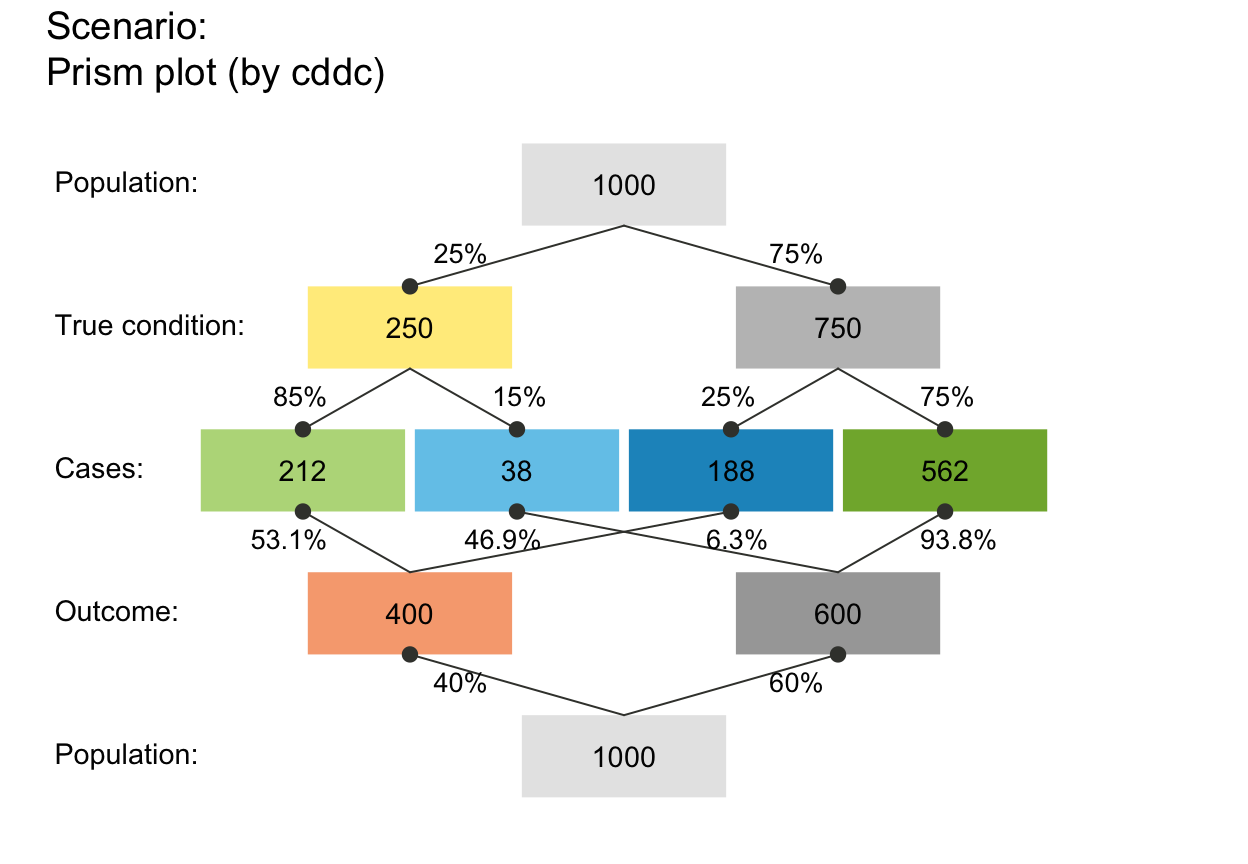plot_prism(f_lwd =  3)  # thicker lines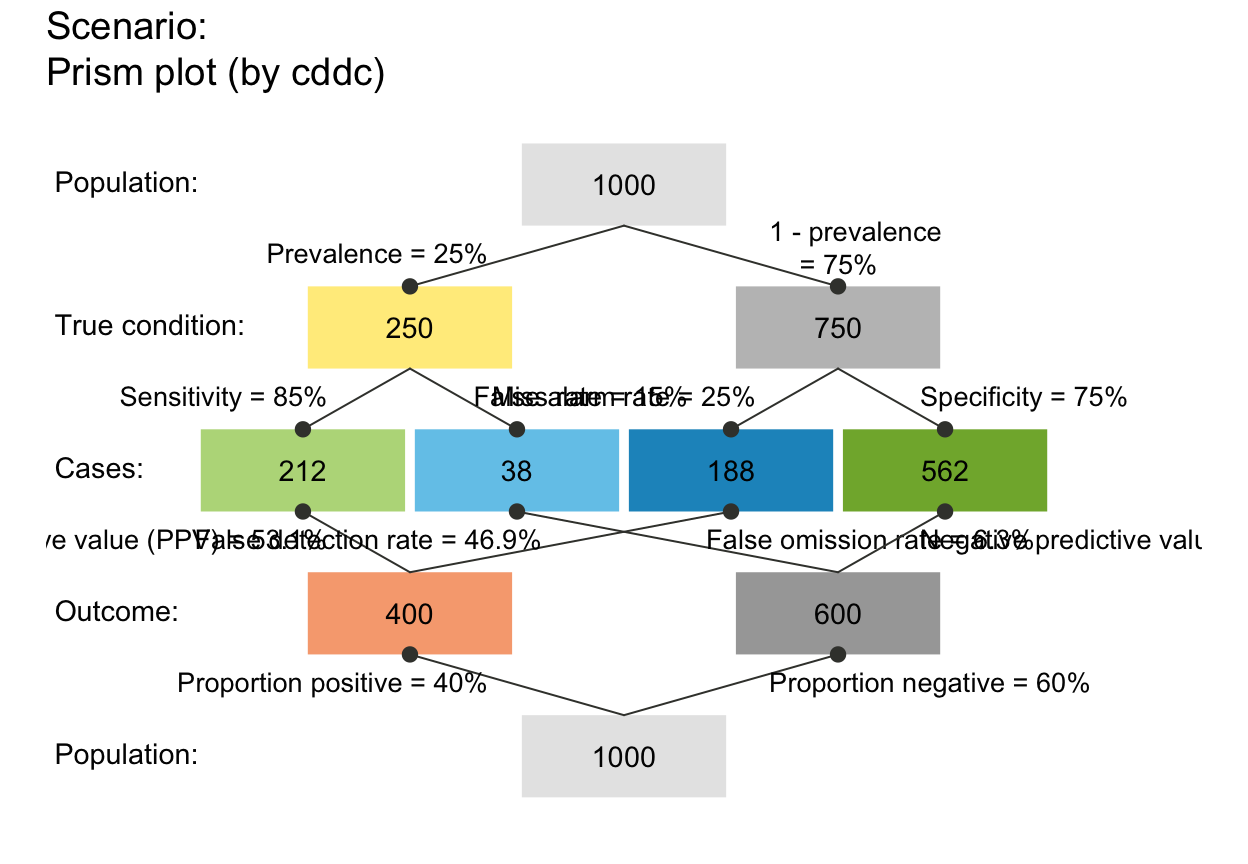plot_prism(f_lwd = .5)  # thinner lines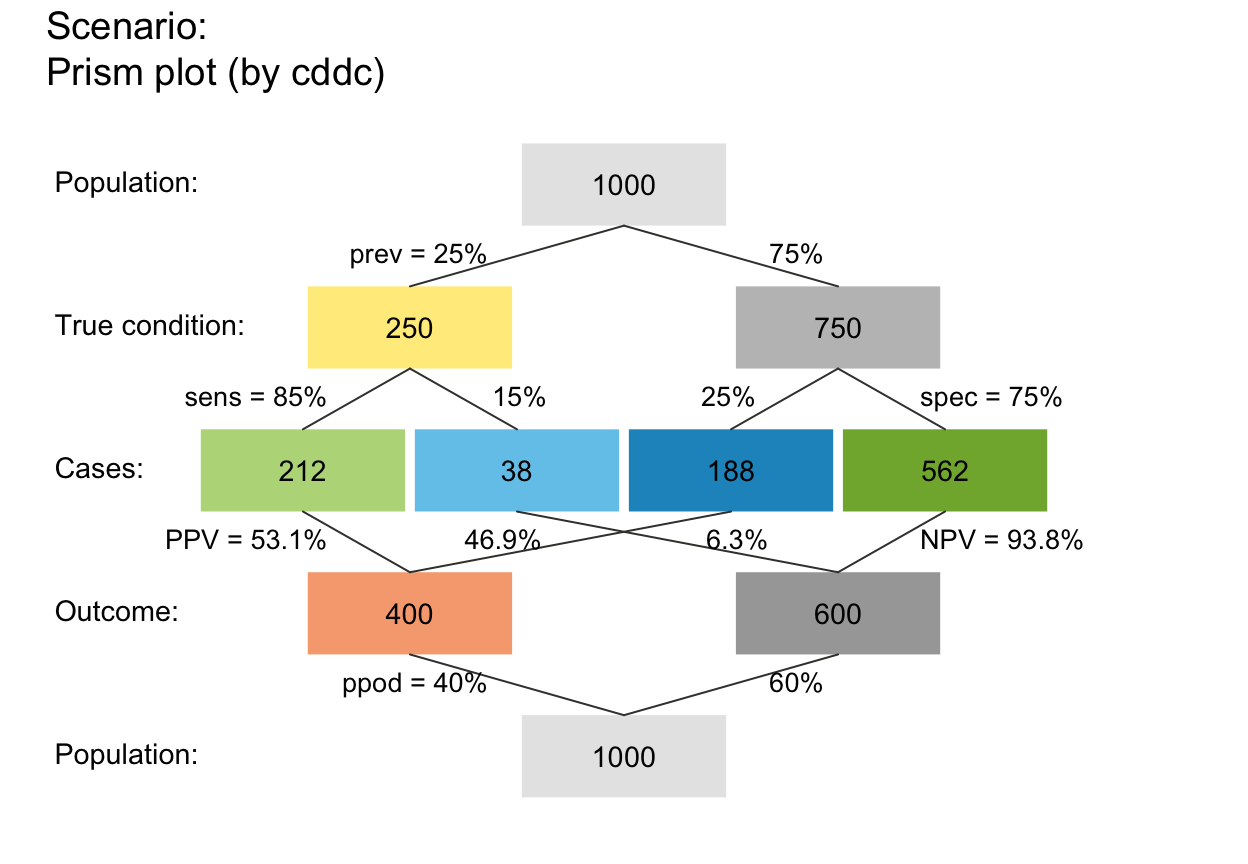# Scale link widths (p_lwd & p_scale):
plot_prism(p_lwd = 6, p_scale = TRUE)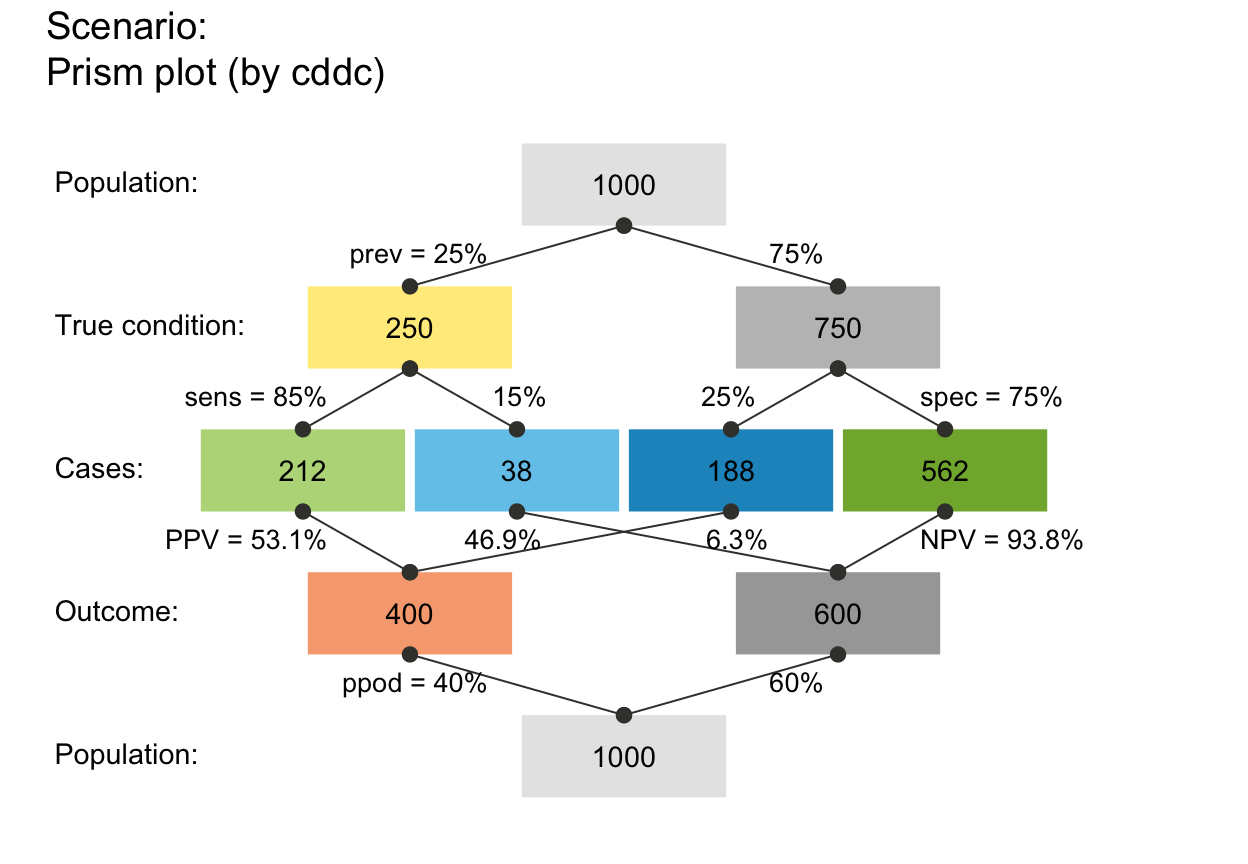plot_prism(area = "sq", f_lbl = "num", p_lbl = NA, col_pal = pal_bw, p_lwd = 6, p_scale = TRUE)plot_prism(area = "hr", f_lbl = "num", f_lwd = .5, p_lbl = NA, arr_c = 0,
col_pal = pal_mod, p_lwd = 6, p_scale = TRUE)# p_lbl:
plot_prism(p_lbl = "mix")     # abbreviated names with numeric values (abb = num)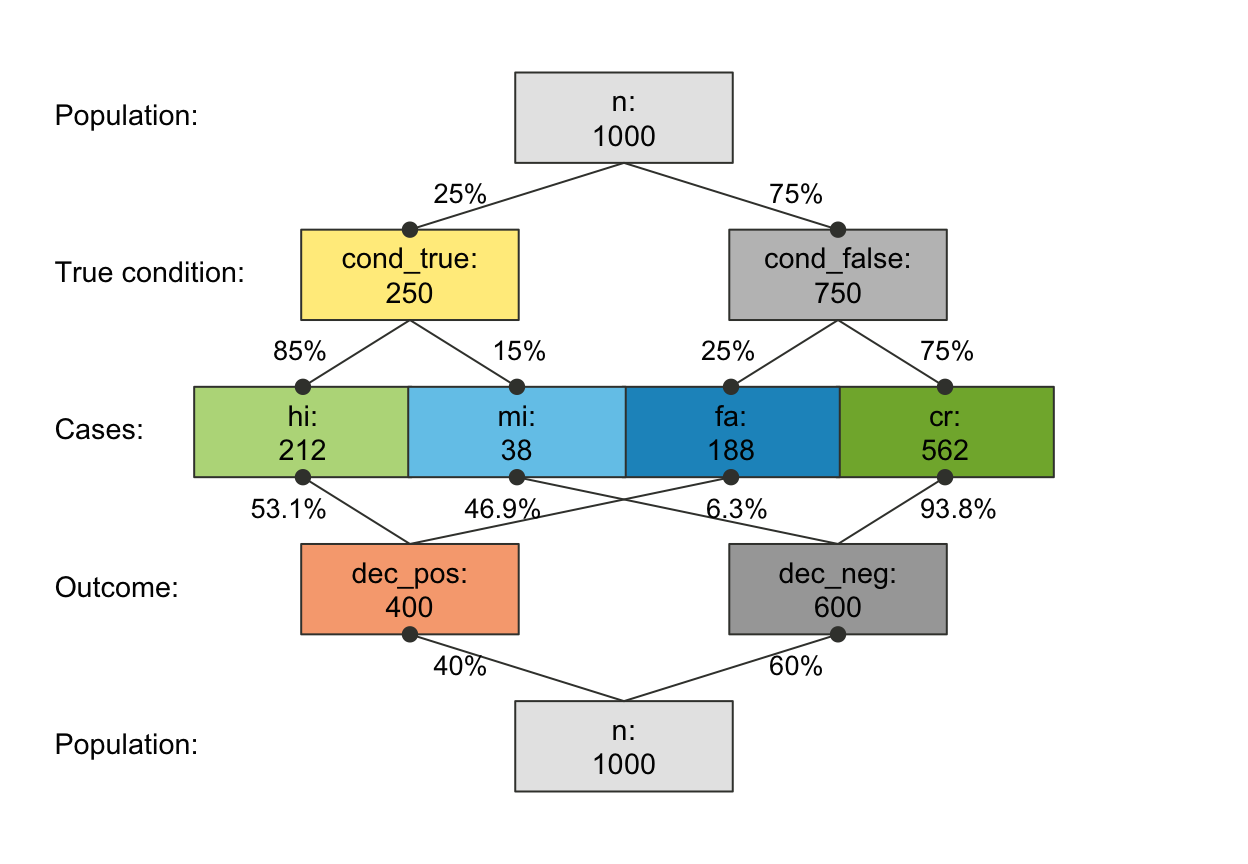plot_prism(p_lbl = "min")     # minimal names (of key probabilities)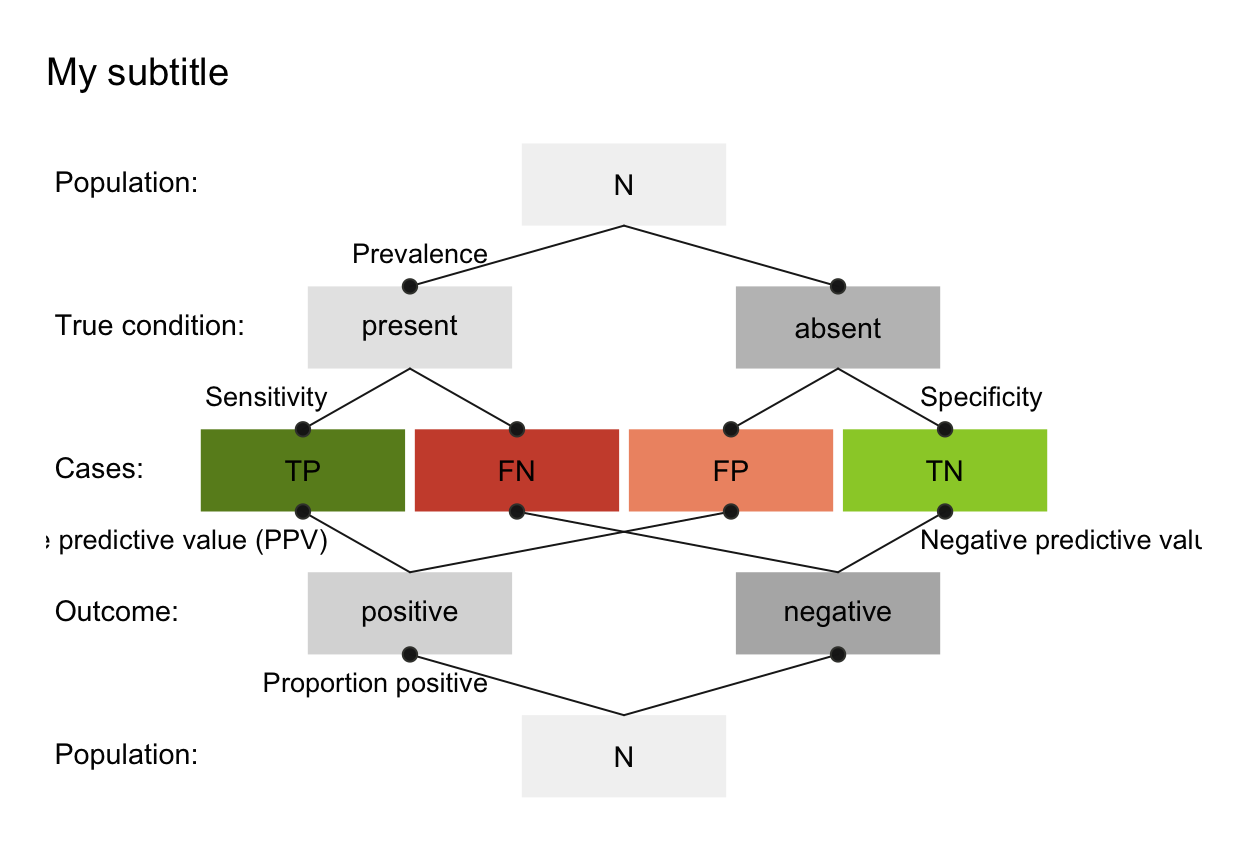plot_prism(p_lbl = NA)        # no prob labels (NA/NULL/"none")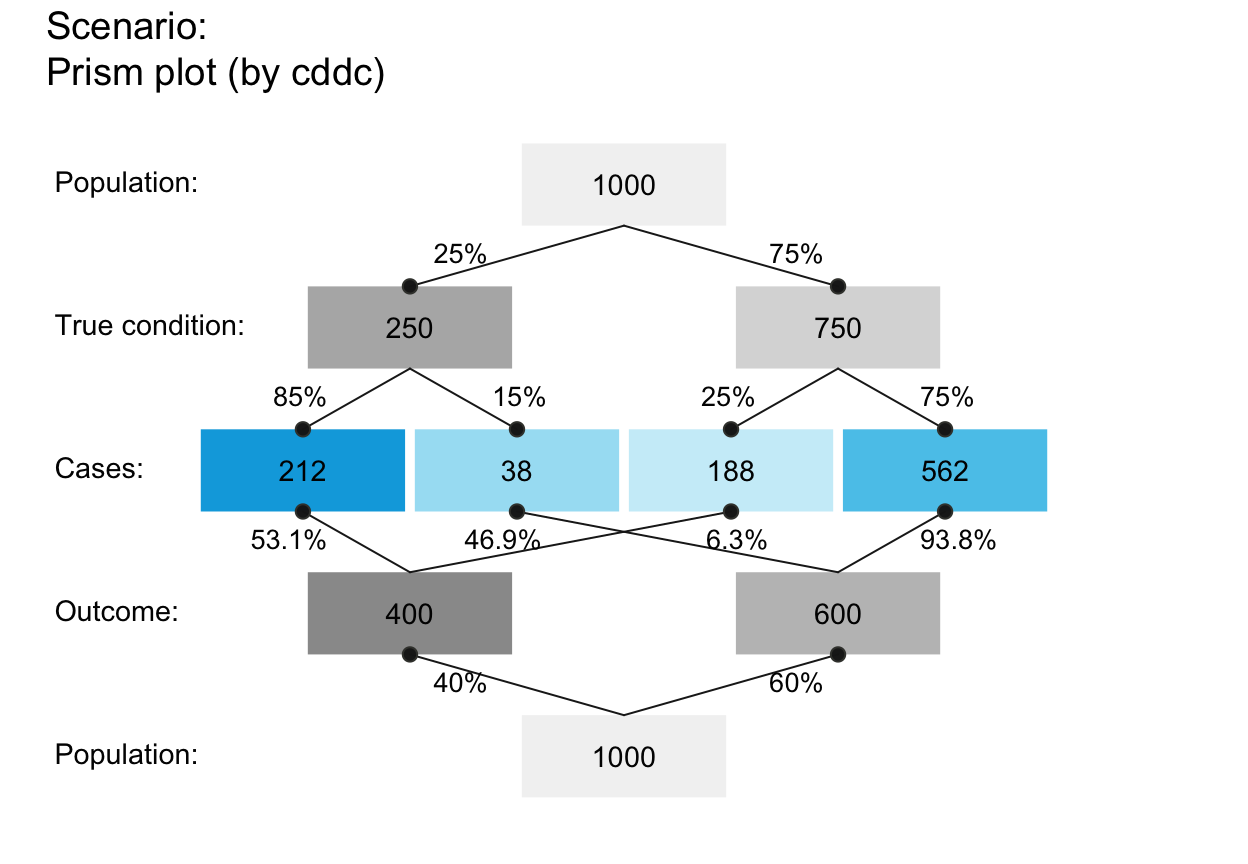plot_prism(p_lbl = "nam")     # only prob names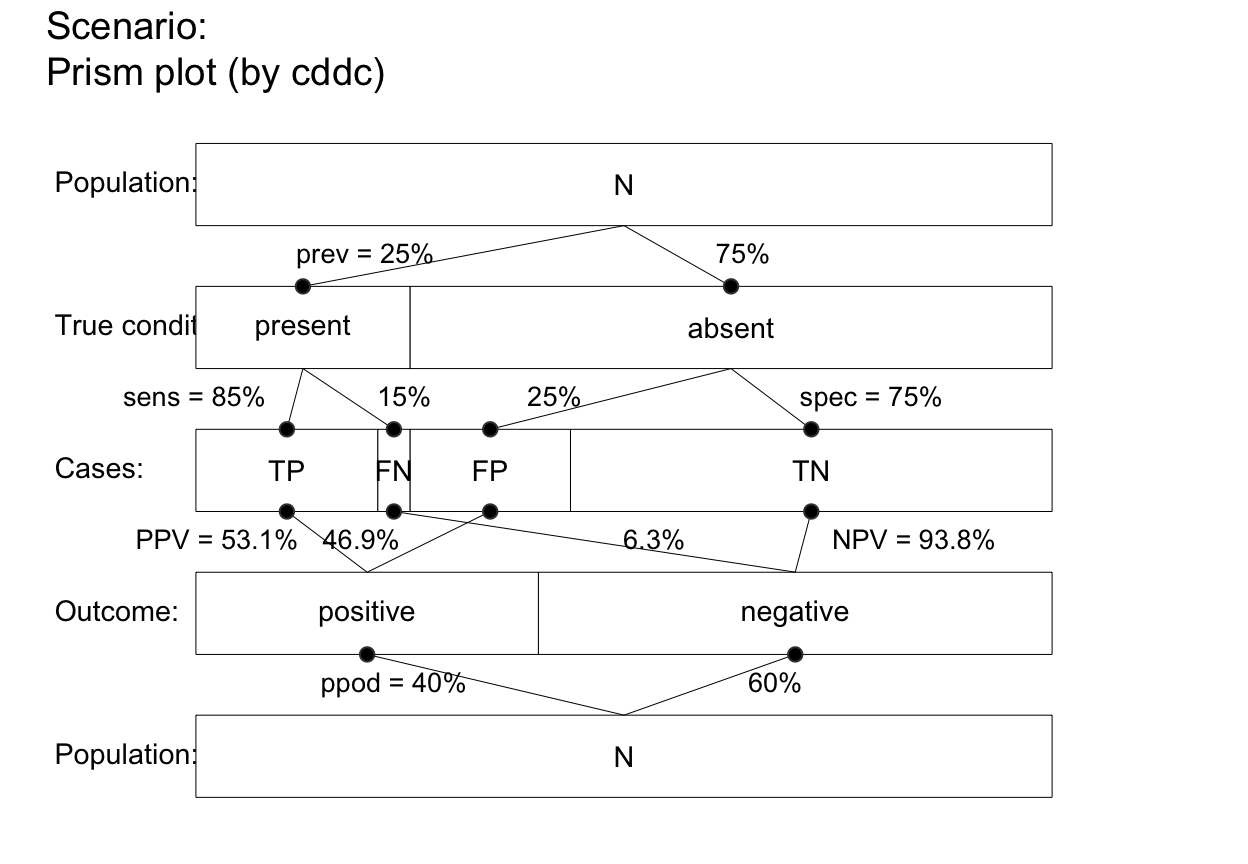plot_prism(p_lbl = "num")     # only numeric prob valuesplot_prism(p_lbl = "namnum")  # names and numeric prob values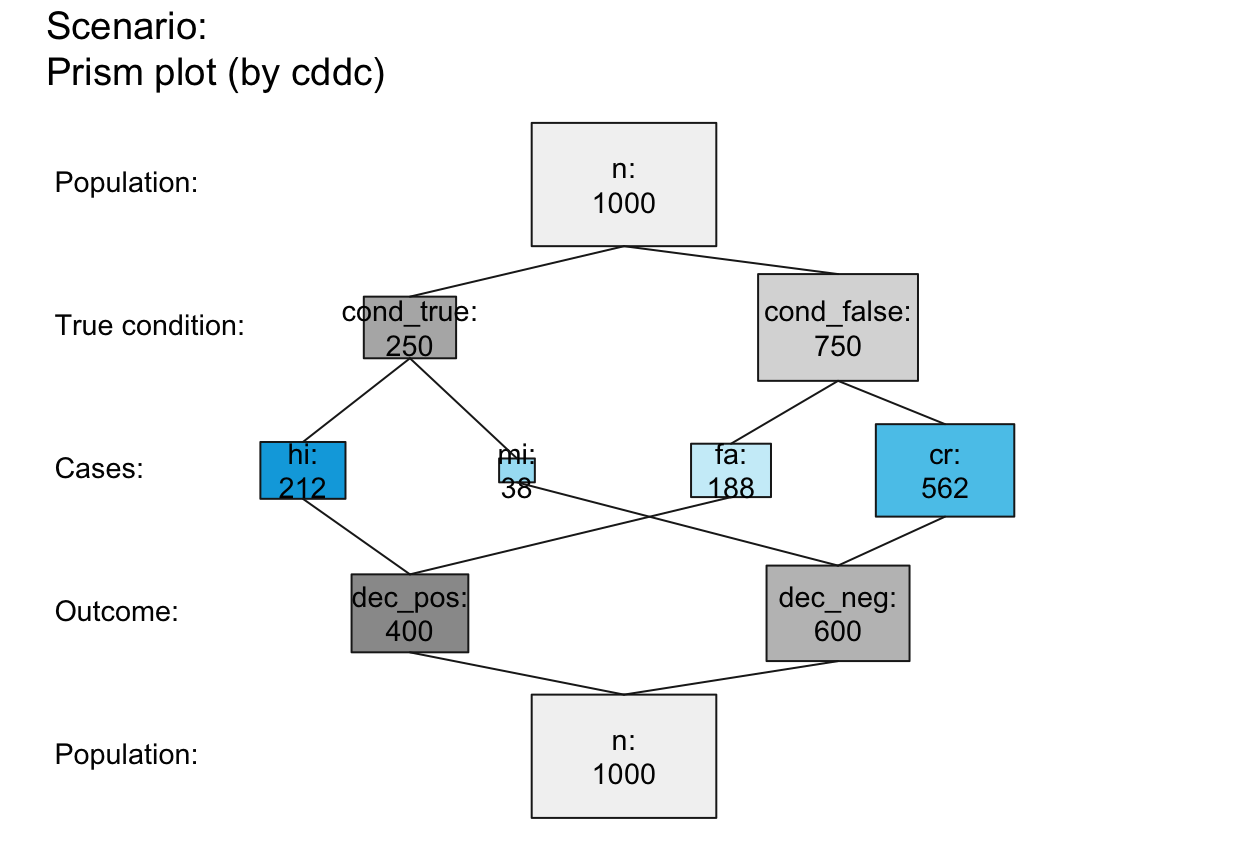# plot_prism(p_lbl = "namnum", cex_p_lbl = .70)  # smaller prob labels
# plot_prism(by = "cddc", p_lbl = "min")  # minimal labels
# plot_prism(by = "cdac", p_lbl = "min")
# plot_prism(by = "cddc", p_lbl = "mix")  # mix abbreviated names and numeric values
# plot_prism(by = "cdac", p_lbl = "mix")
# plot_prism(by = "cddc", p_lbl = "abb")  # abbreviated names
# plot_prism(by = "cdac", p_lbl = "abb")
# plot_prism(p_lbl = "any") # short name and value (abb = num)

# arr_c:
plot_prism(arr_c =  0)  # acc_c = 0: no arrowsplot_prism(arr_c = -3)  # arr_c = -1 to -3: points at both endsplot_prism(arr_c = -2)  # point at far end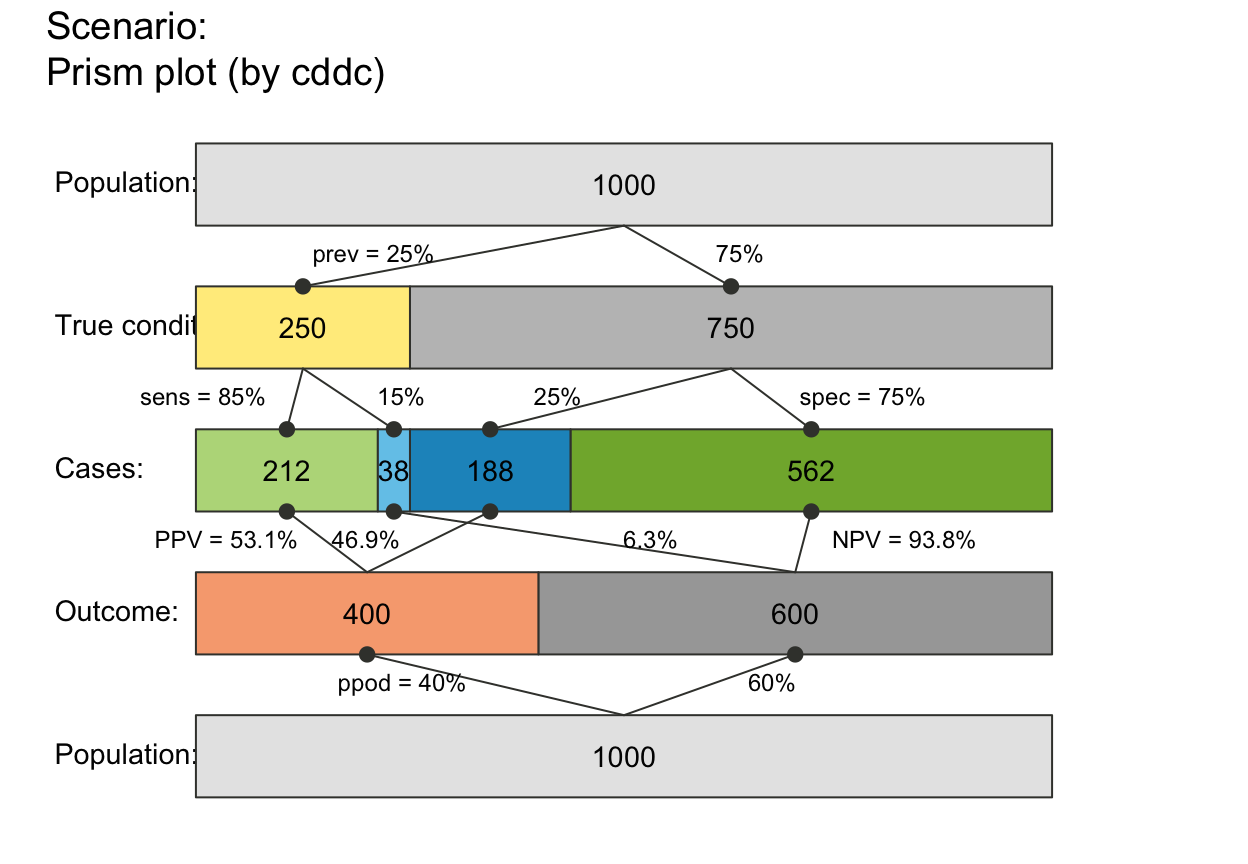plot_prism(arr_c = +2)  # crr_c = 1-3: V-shape arrows at far end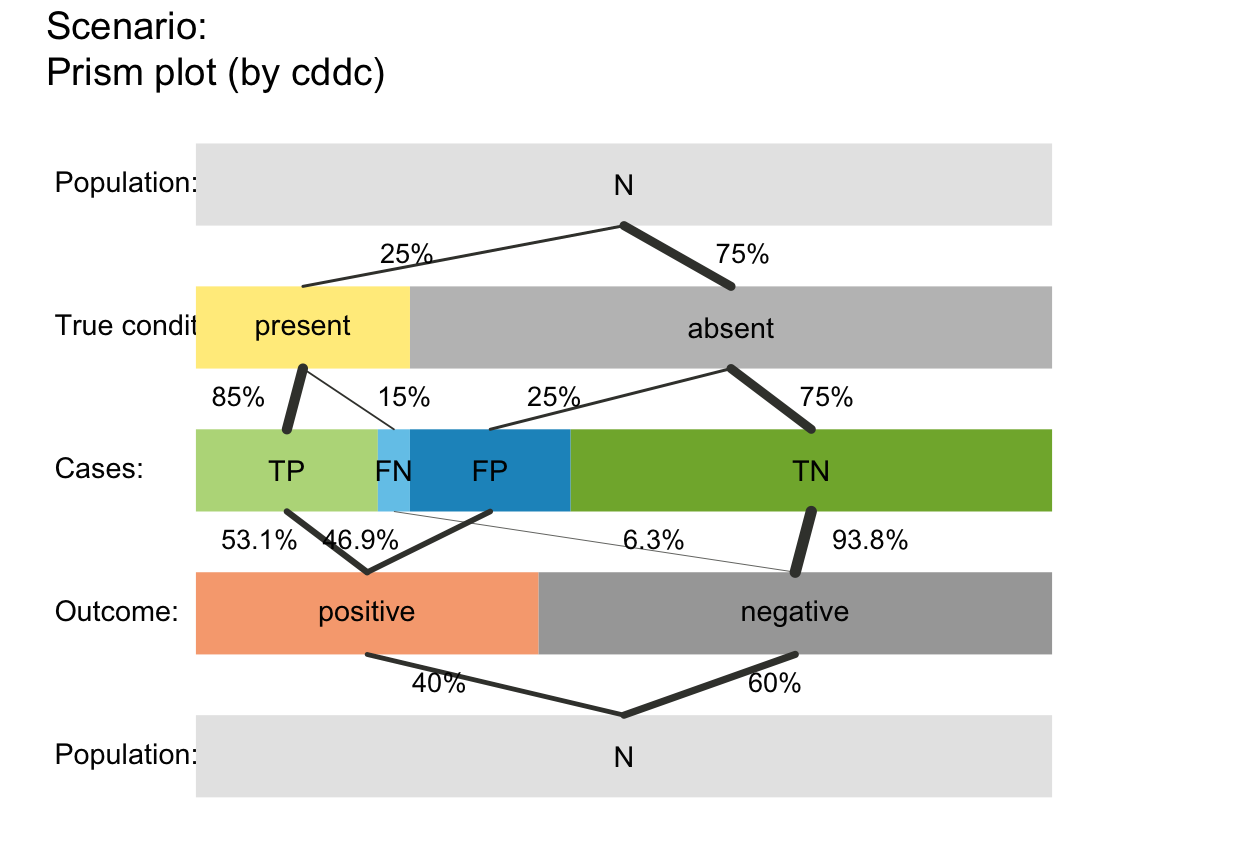# plot_prism(arr_c = +3)  # V-shape arrows at both ends
# plot_prism(arr_c = +6)  # arr_c = 4-6: T-shape arrows

## Plain plot versions:
plot_prism(area = "no", f_lbl = "def", p_lbl = "num", col_pal = pal_mod, f_lwd = 1,
title_lbl = "", mar_notes = FALSE)  # remove titles and margin notes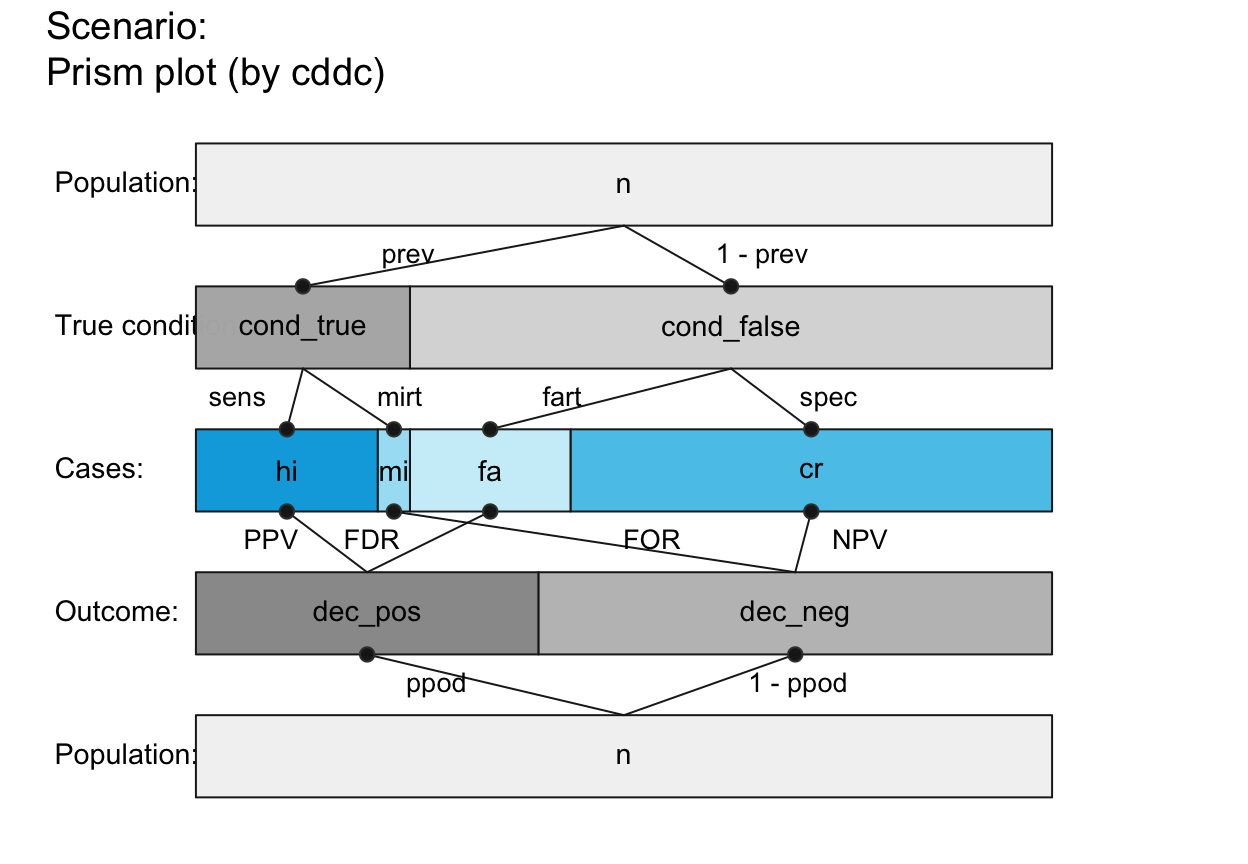plot_prism(area = "no", f_lbl = "nam", p_lbl = "min", col_pal = pal_rgb)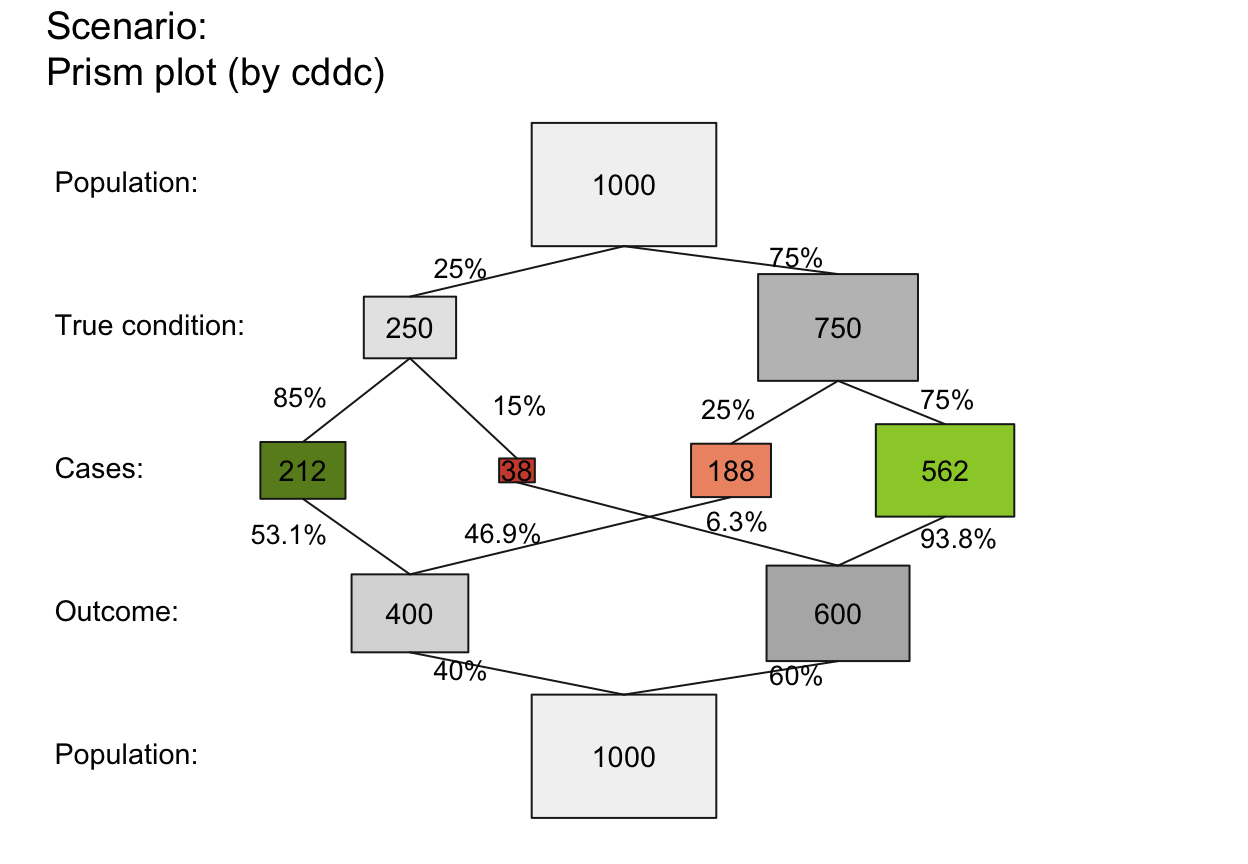plot_prism(area = "no", f_lbl = "num", p_lbl = "num", col_pal = pal_kn)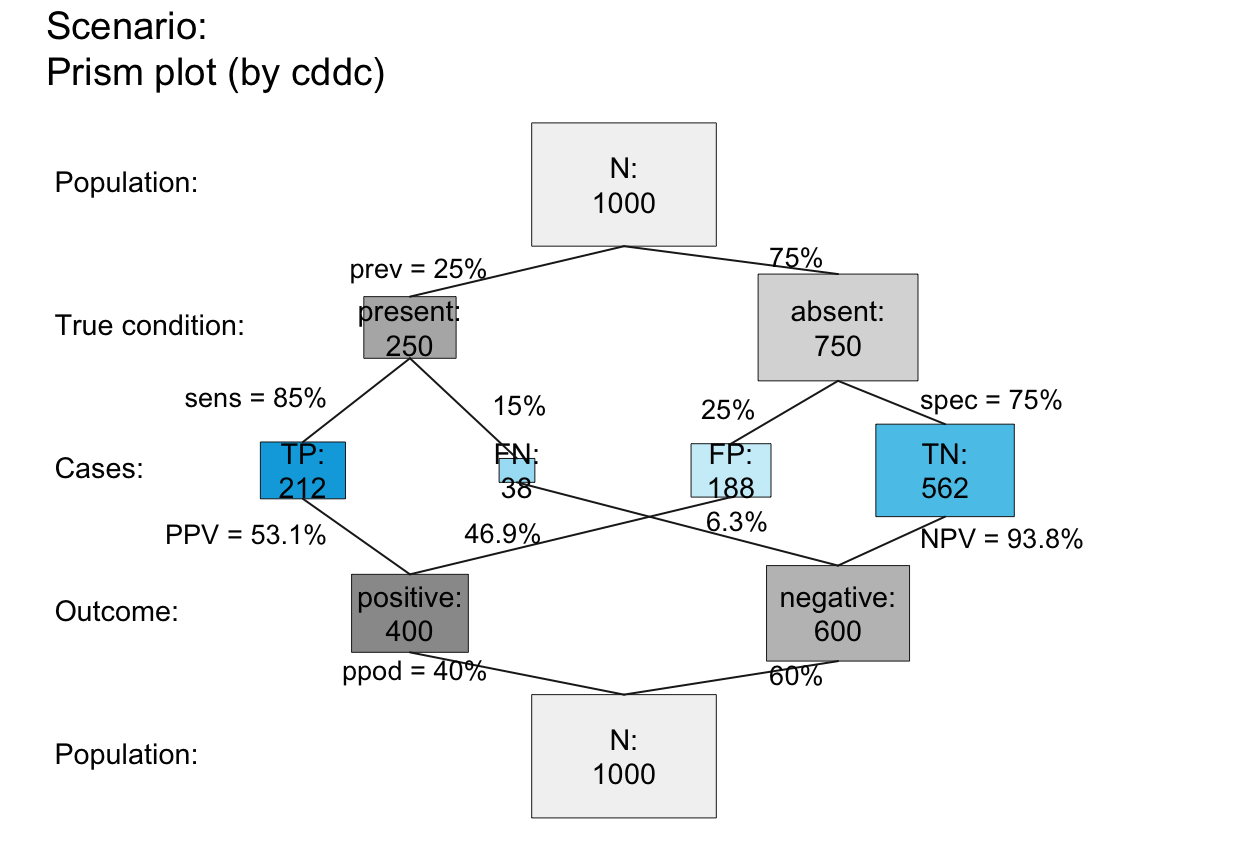plot_prism(area = "hr", f_lbl = "nam", f_lwd = .5, p_lwd = .5, col_pal = pal_bwp)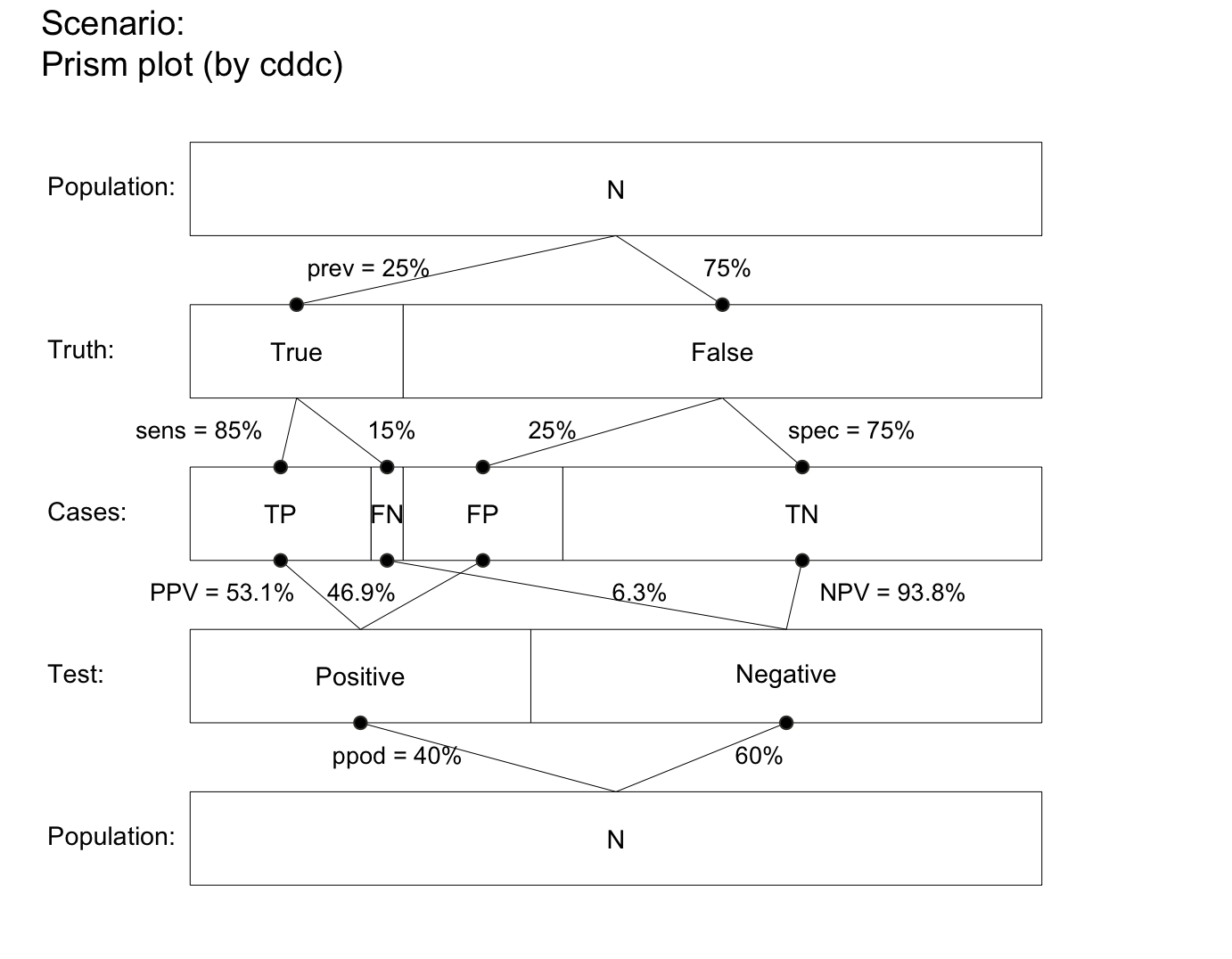plot_prism(area = "hr", f_lbl = "nam", f_lwd = .5, p_lbl = "num")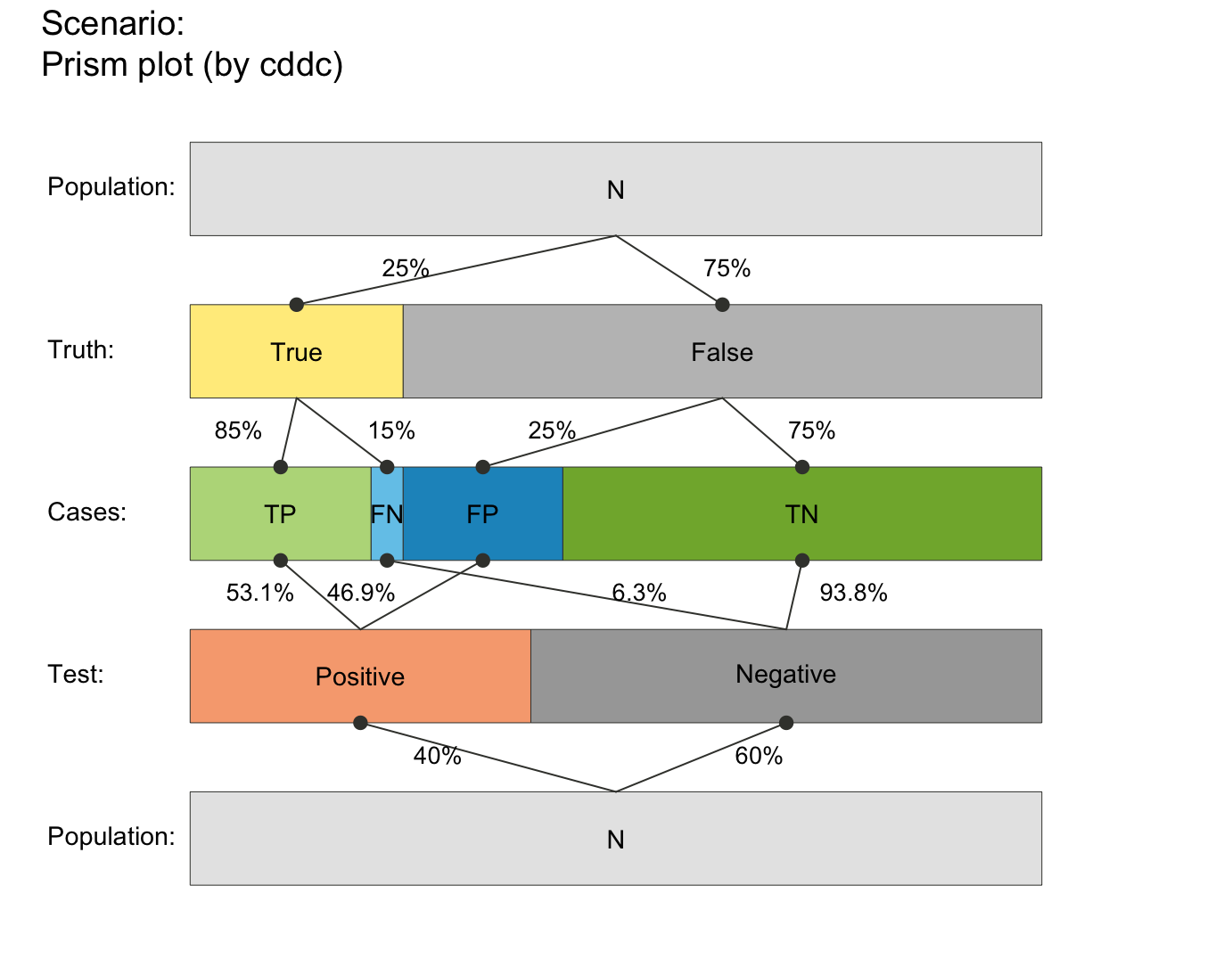plot_prism(area = "sq", f_lbl = "nam", p_lbl = NA, col_pal = pal_rgb)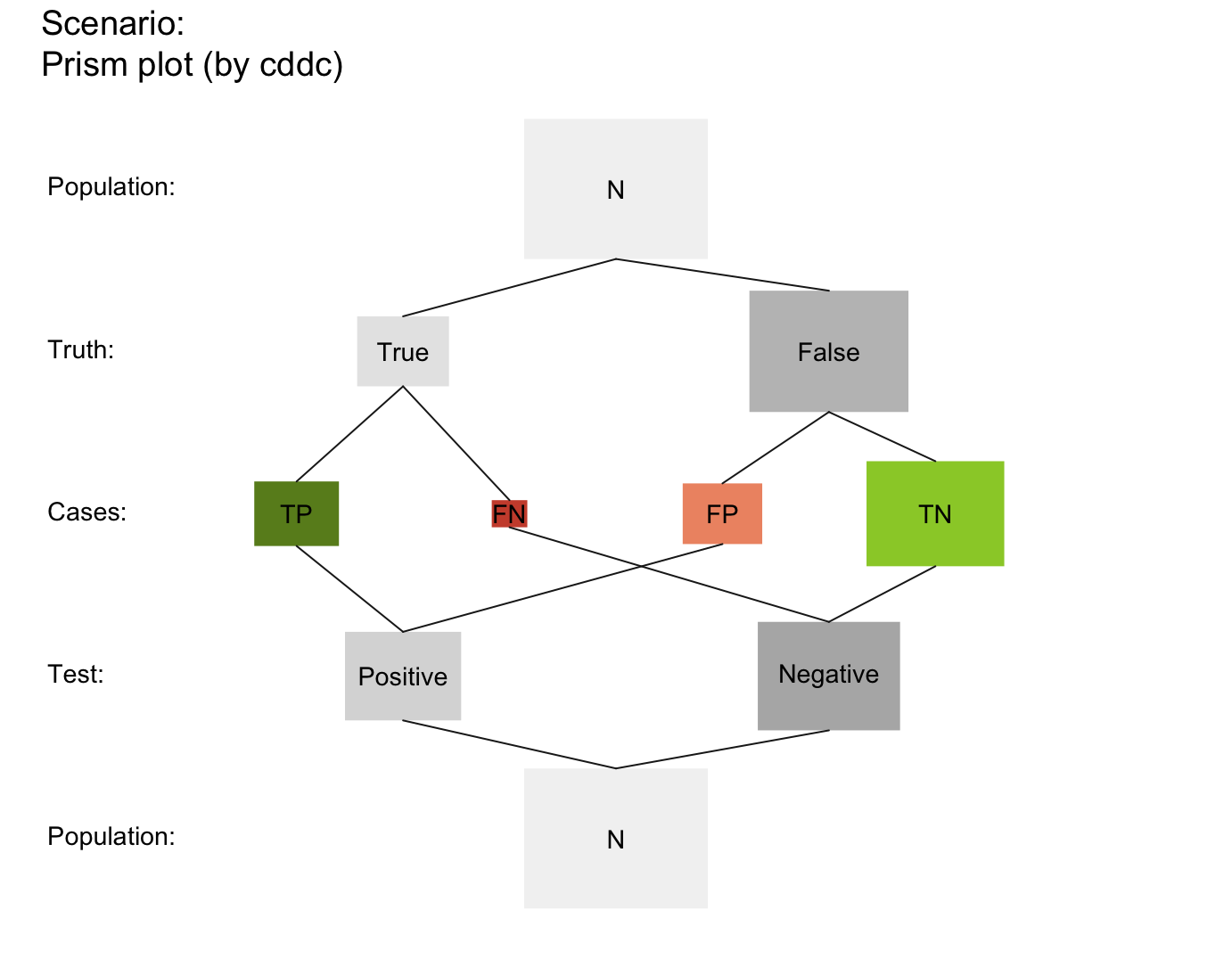plot_prism(area = "sq", f_lbl = "def", f_lbl_sep = ":\n", p_lbl = NA, f_lwd = 1, col_pal = pal_kn)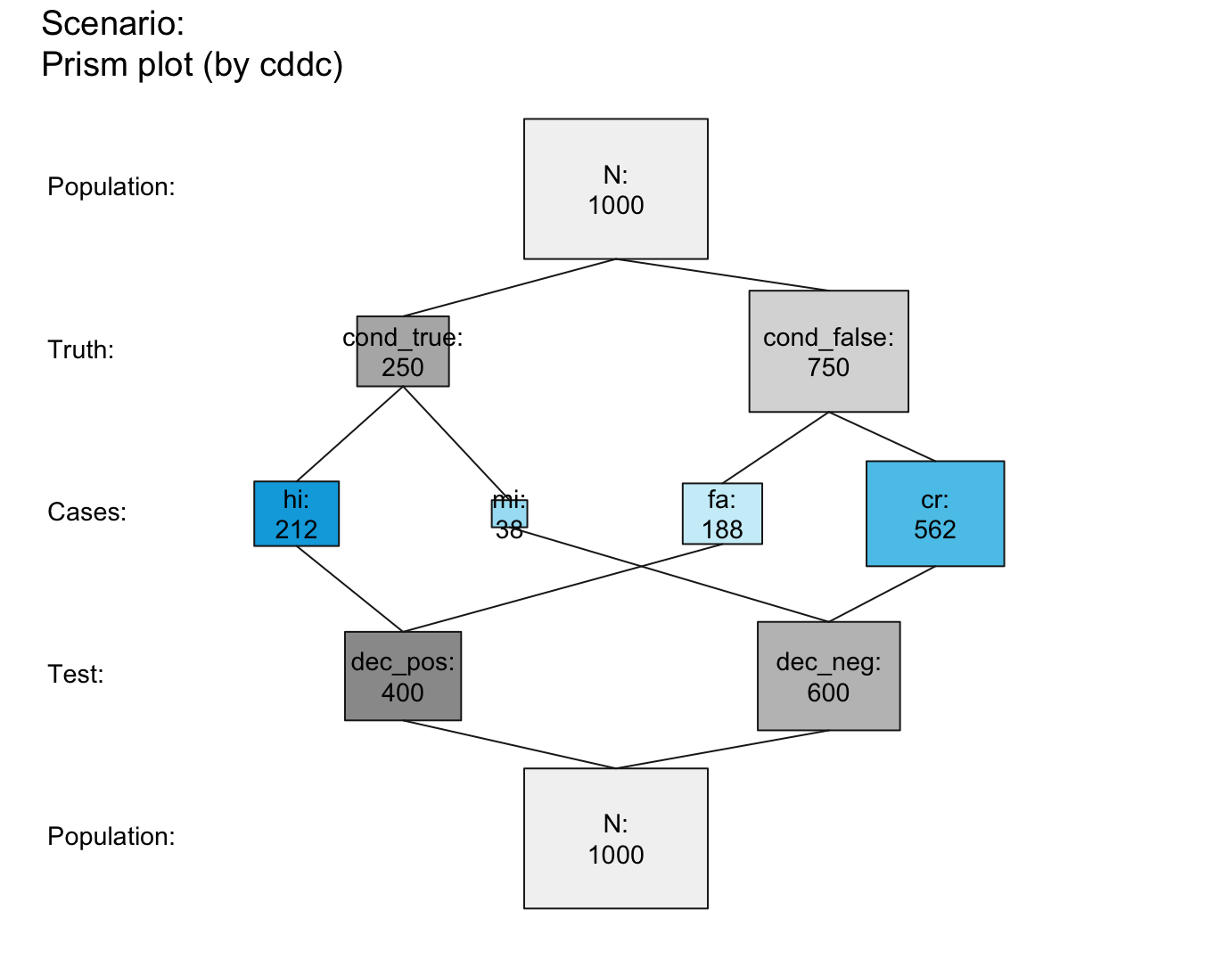## Suggested combinations:
plot_prism(f_lbl = "nam", p_lbl = "mix", col_pal = pal_mod)  # basic plot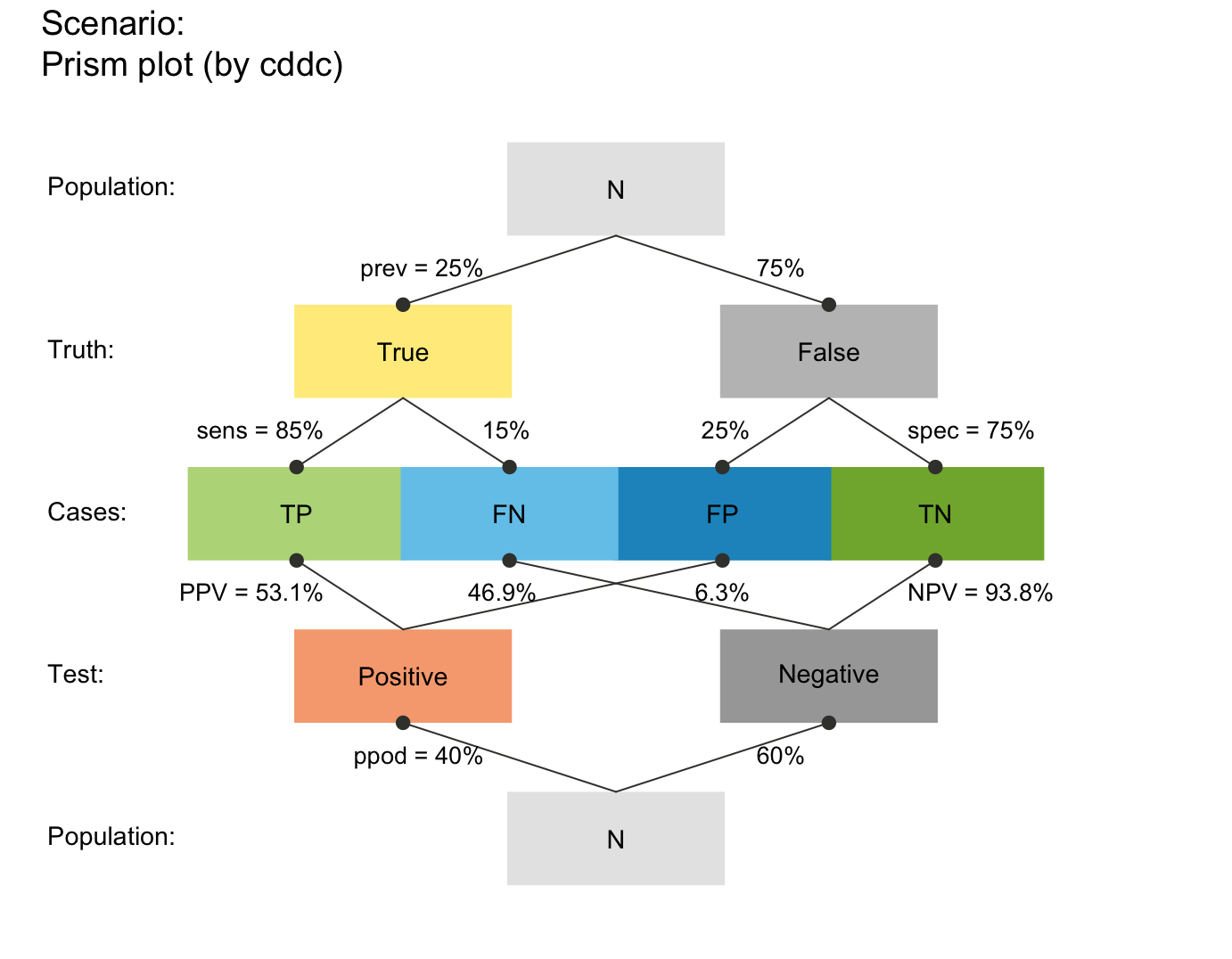plot_prism(f_lbl = "namnum", p_lbl = "num", cex_lbl = .80, cex_p_lbl = .75)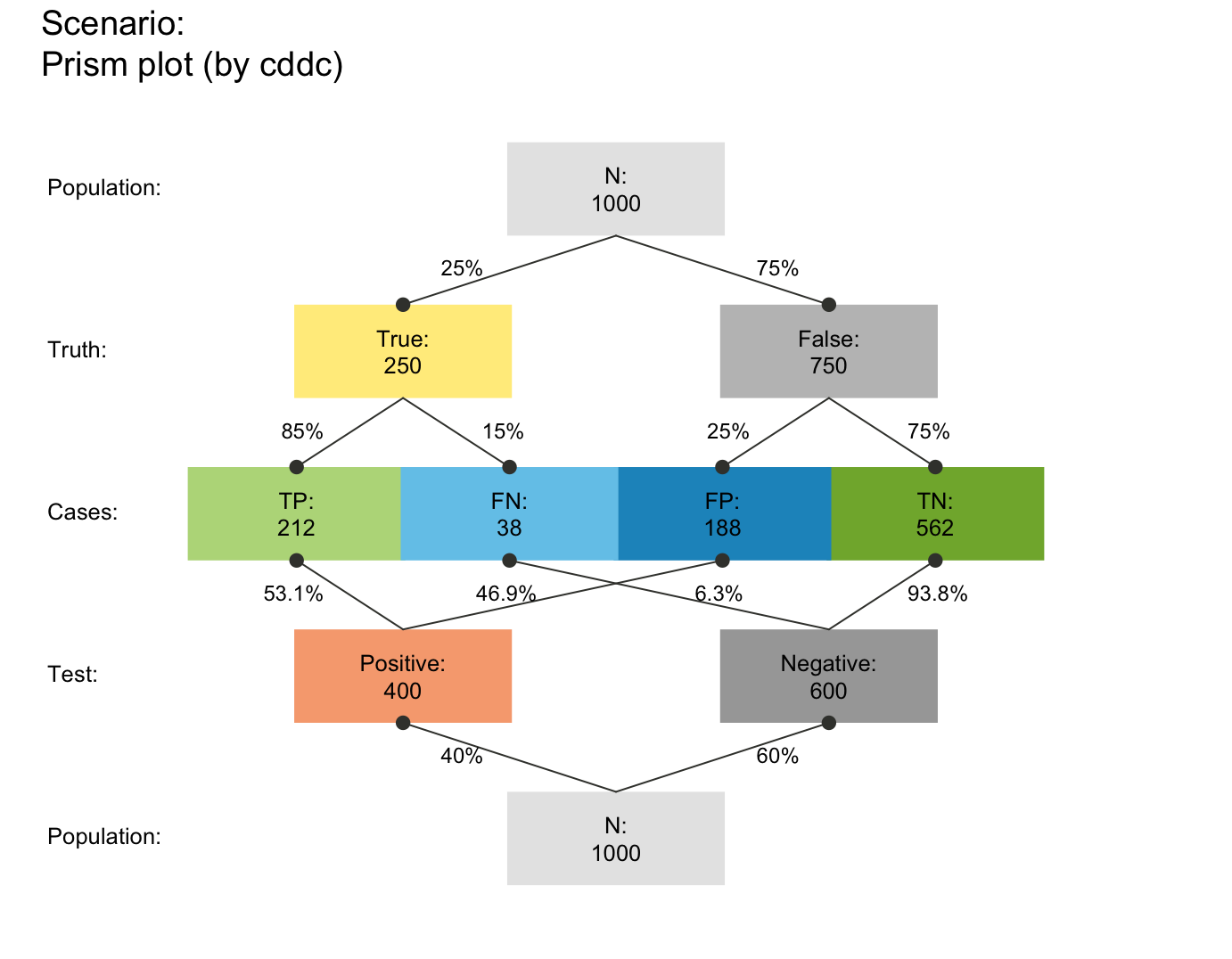plot_prism(area = "no", f_lbl = "def", p_lbl = "abb",           # def/abb labels
f_lwd = .8, p_lwd = .8, lty = 3, col_pal = pal_bwp)  # black-&-white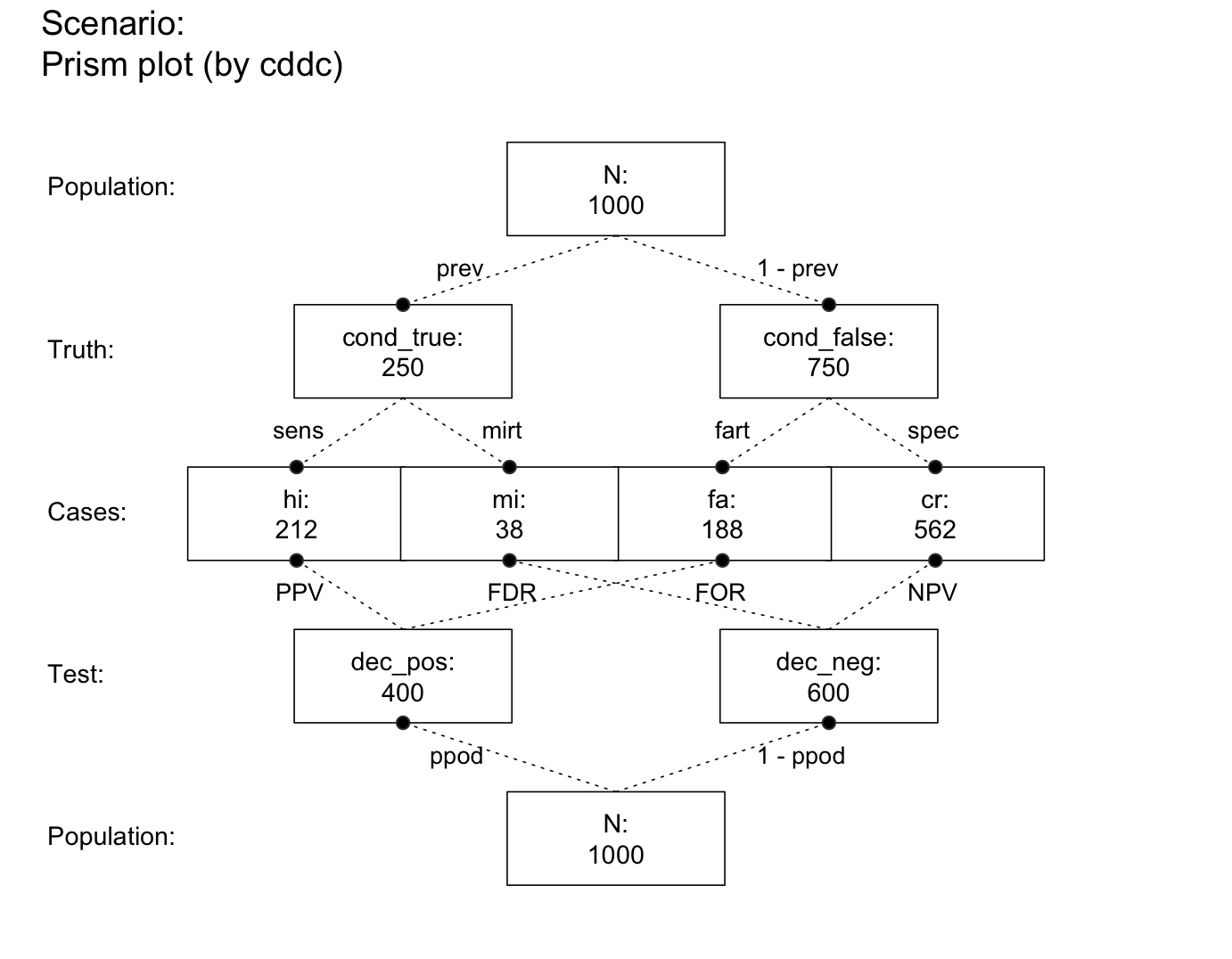plot_prism(area = "hr", f_lbl = "num", p_lbl = "mix", f_lwd = 1, cex_p_lbl = .75)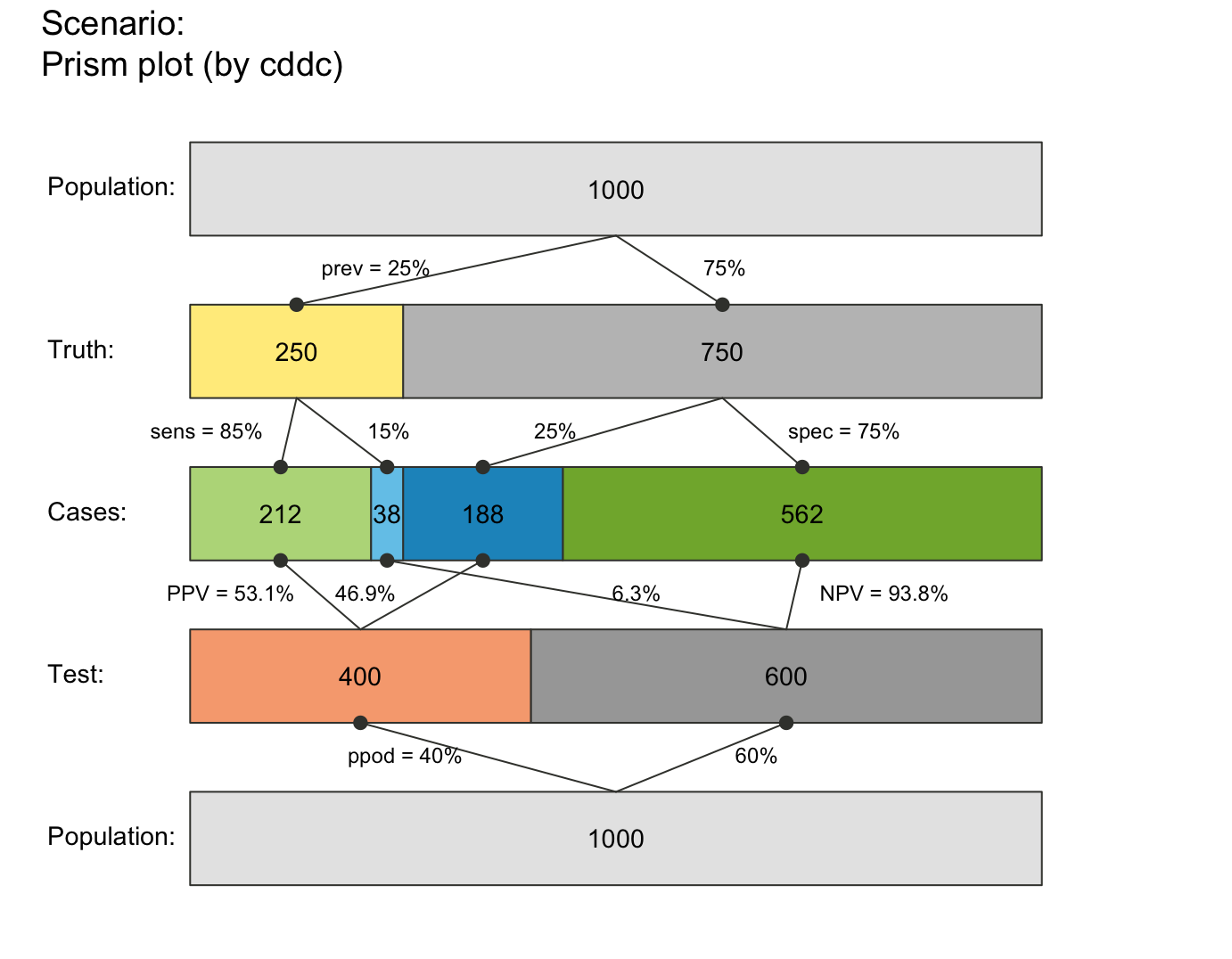plot_prism(area = "hr", f_lbl = "nam", p_lbl = "num", p_lwd = 6, p_scale = TRUE)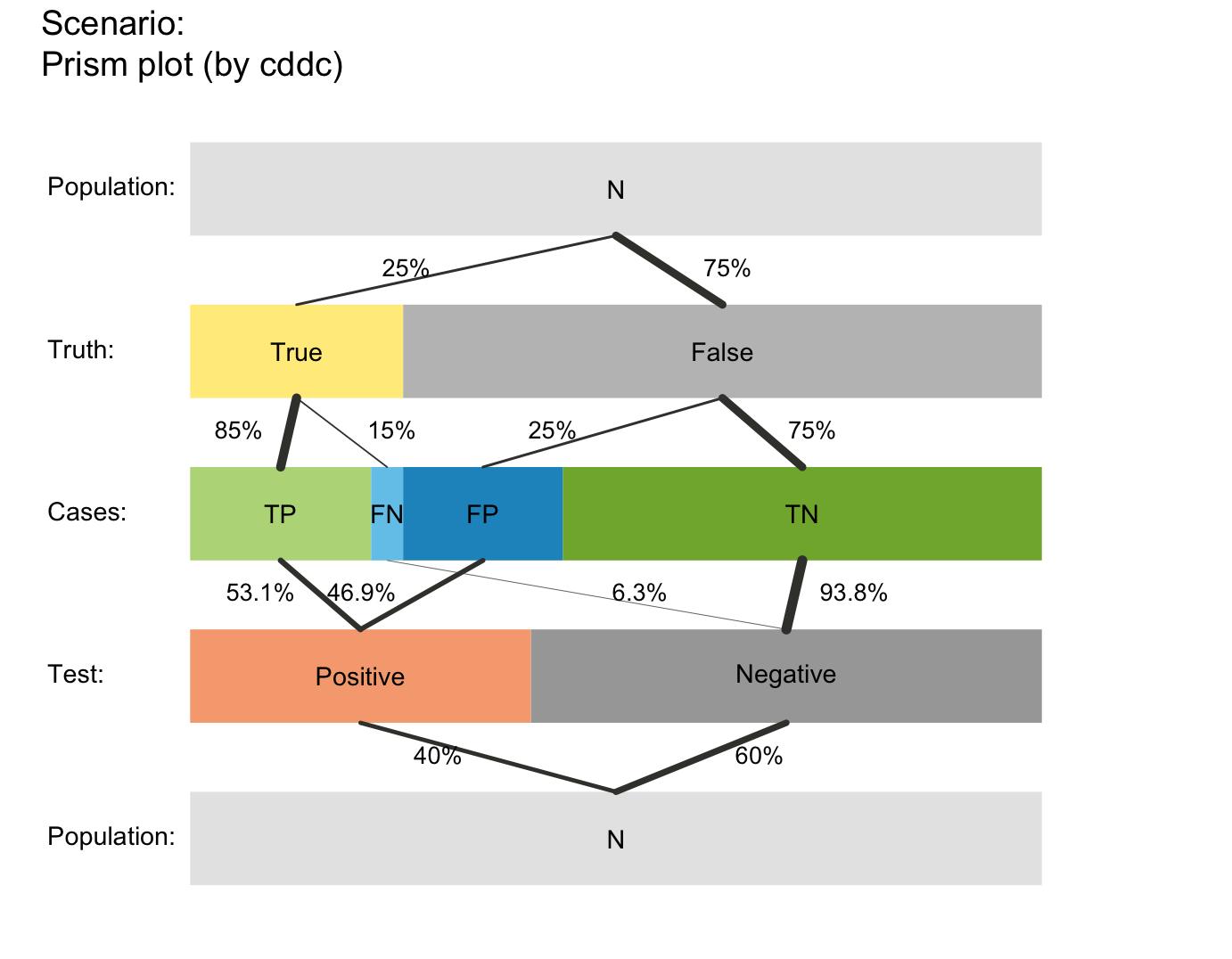plot_prism(area = "hr", f_lbl = "abb", p_lbl = "abb", f_lwd = 1, col_pal = pal_kn)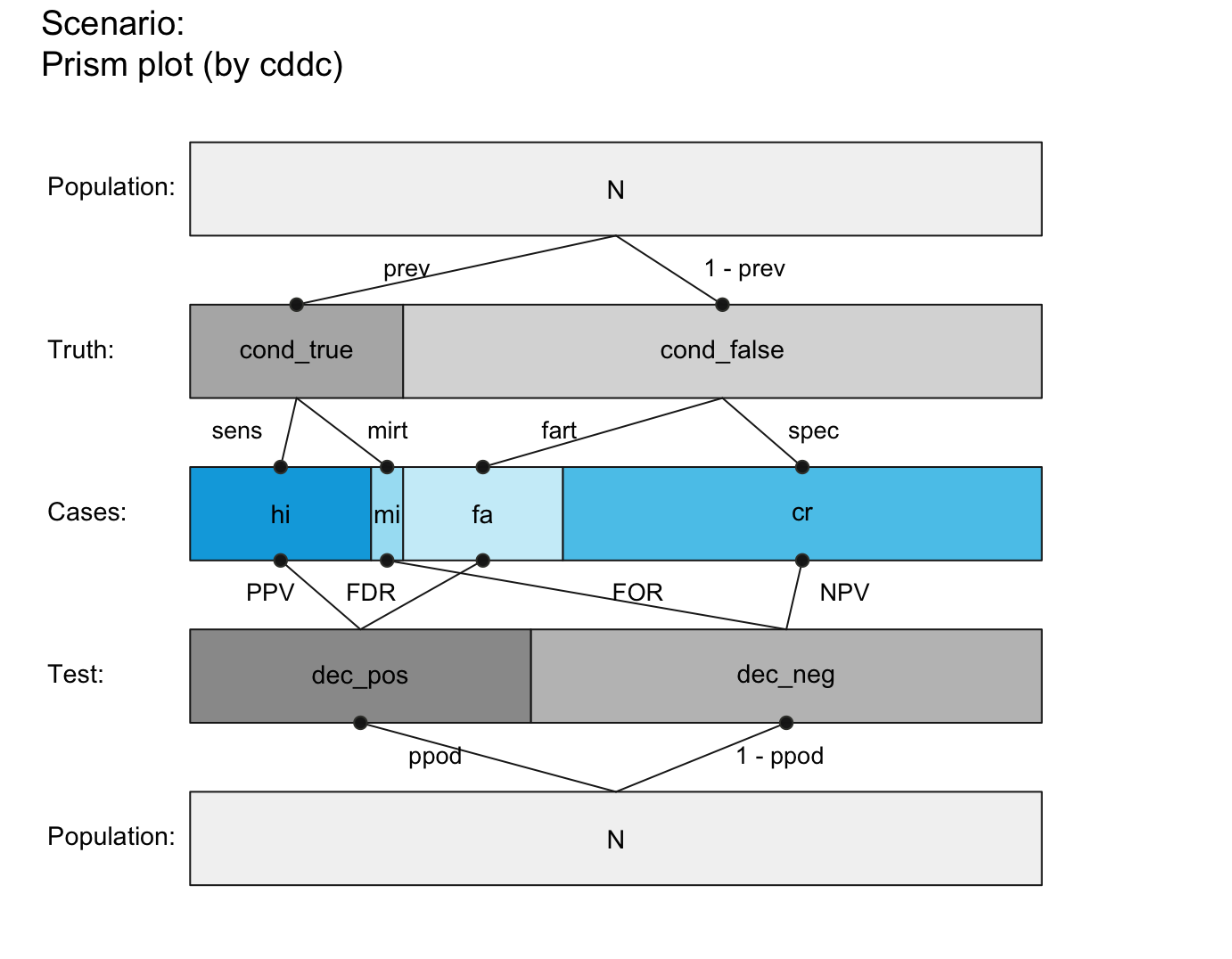plot_prism(area = "sq", f_lbl = "nam", p_lbl = "abb", lbl_txt = txt_TF)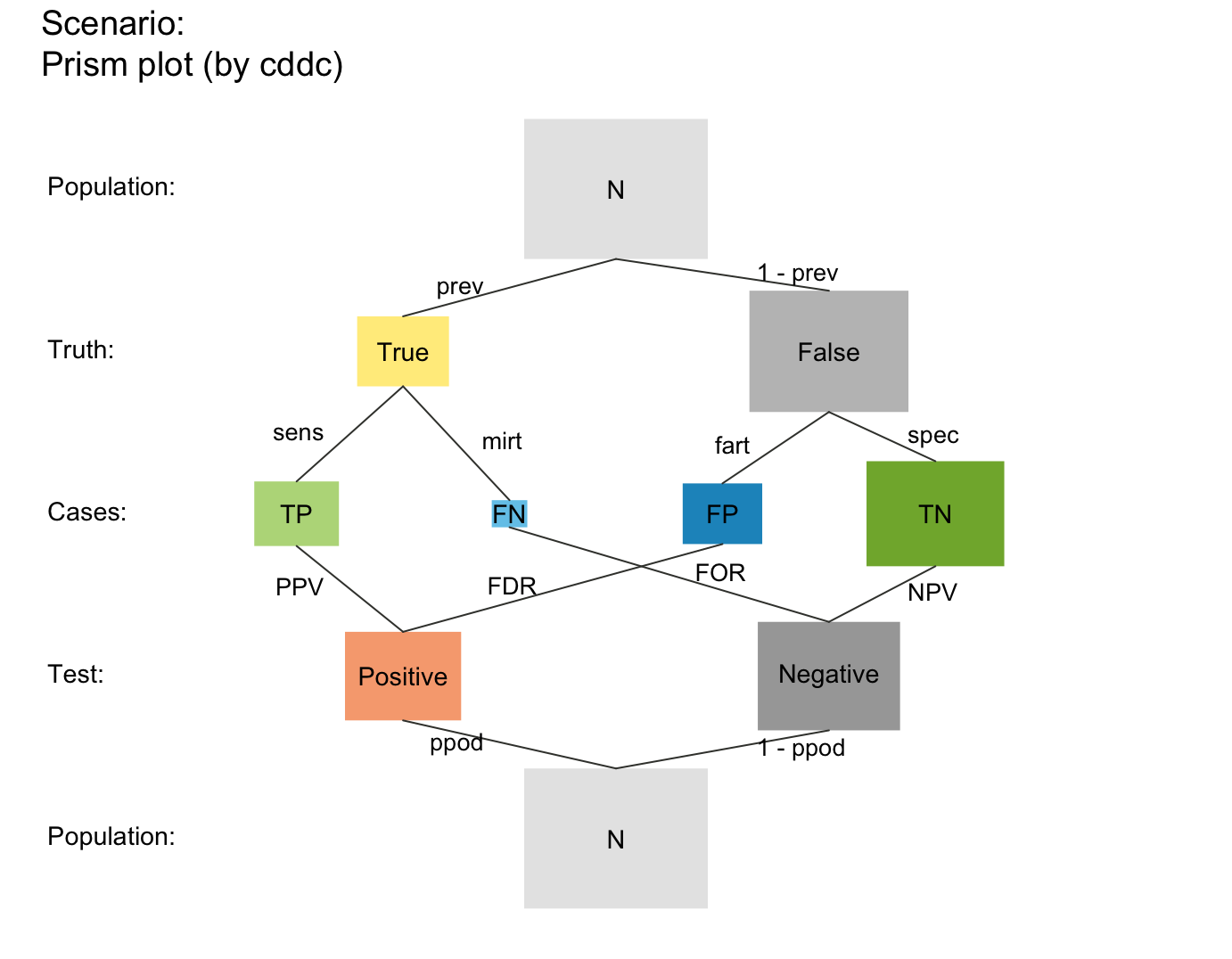plot_prism(area = "sq", f_lbl = "num", p_lbl = "num", f_lwd = 1, col_pal = pal_rgb)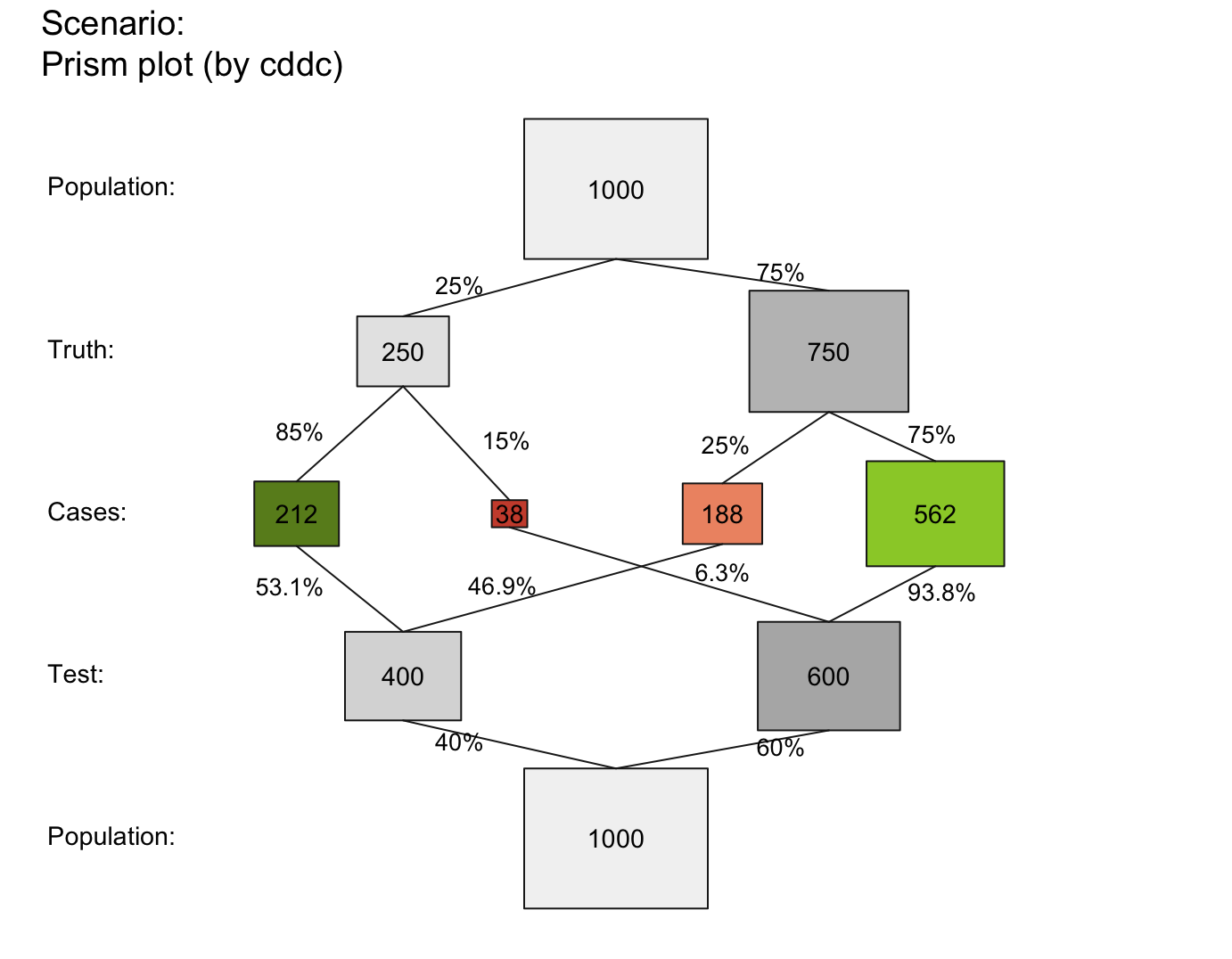plot_prism(area = "sq", f_lbl = "namnum", p_lbl = "mix", f_lwd = .5, col_pal = pal_kn)